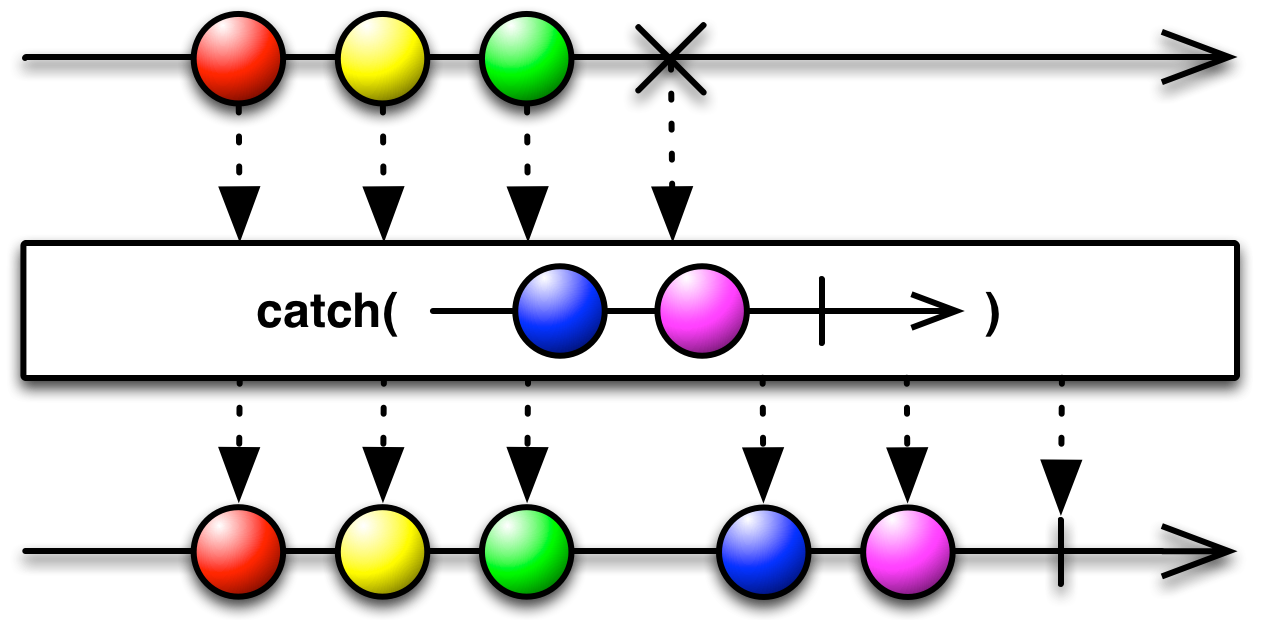Module

# RxJS.Observable

Package
purescript-rxps
Repository
LukaJCB/purescript-rxps

### #ObservableImplSource

``data ObservableImpl :: Type -> Type``

#### Instances

• `Monoid (ObservableImpl a)`
• `Functor ObservableImpl`
• `Apply ObservableImpl`
• `Applicative ObservableImpl`
• `Bind ObservableImpl`
• `Monad ObservableImpl`
• `Semigroup (ObservableImpl a)`
• `Alt ObservableImpl`
• `Plus ObservableImpl`
• `Alternative ObservableImpl`
• `MonadZero ObservableImpl`
• `MonadPlus ObservableImpl`
• `MonadError Error ObservableImpl`
• `MonadThrow Error ObservableImpl`
• `(Arbitrary a) => Arbitrary (ObservableImpl a)`

### #ResponseSource

``type Response = { body :: String, responseType :: String, status :: Int }``

### #RequestSource

``type Request = { body :: String, crossDomain :: Boolean, headers :: StrMap String, method :: String, responseType :: String, timeout :: Int, url :: String }``

### #ObservableTSource

``newtype ObservableT m a``

#### Constructors

• `ObservableT (m (ObservableImpl a))`

#### Instances

• `(Functor f) => Functor (ObservableT f)`
• `(Apply f) => Apply (ObservableT f)`
• `(Applicative f) => Applicative (ObservableT f)`
• `(Apply f) => Semigroup (ObservableT f a)`
• `(Applicative f) => Monoid (ObservableT f a)`
• `(Apply f) => Alt (ObservableT f)`
• `(Applicative f) => Plus (ObservableT f)`
• `(Monad m) => Bind (ObservableT m)`
• `(Monad m) => Monad (ObservableT m)`
• `(Monad m) => Alternative (ObservableT m)`
• `(Monad m) => MonadZero (ObservableT m)`
• `(Monad m) => MonadPlus (ObservableT m)`

### #ObservableSource

``type Observable a = ObservableT Identity a``

### #runObservableTSource

``runObservableT :: forall a m. ObservableT m a -> m (ObservableImpl a)``

### #combineLatest3Source

``combineLatest3 :: forall f d c b a. Apply f => (a -> b -> c -> d) -> ObservableT f a -> ObservableT f b -> ObservableT f c -> ObservableT f d``

An Observable of projected values from the most recent values from each input Observable.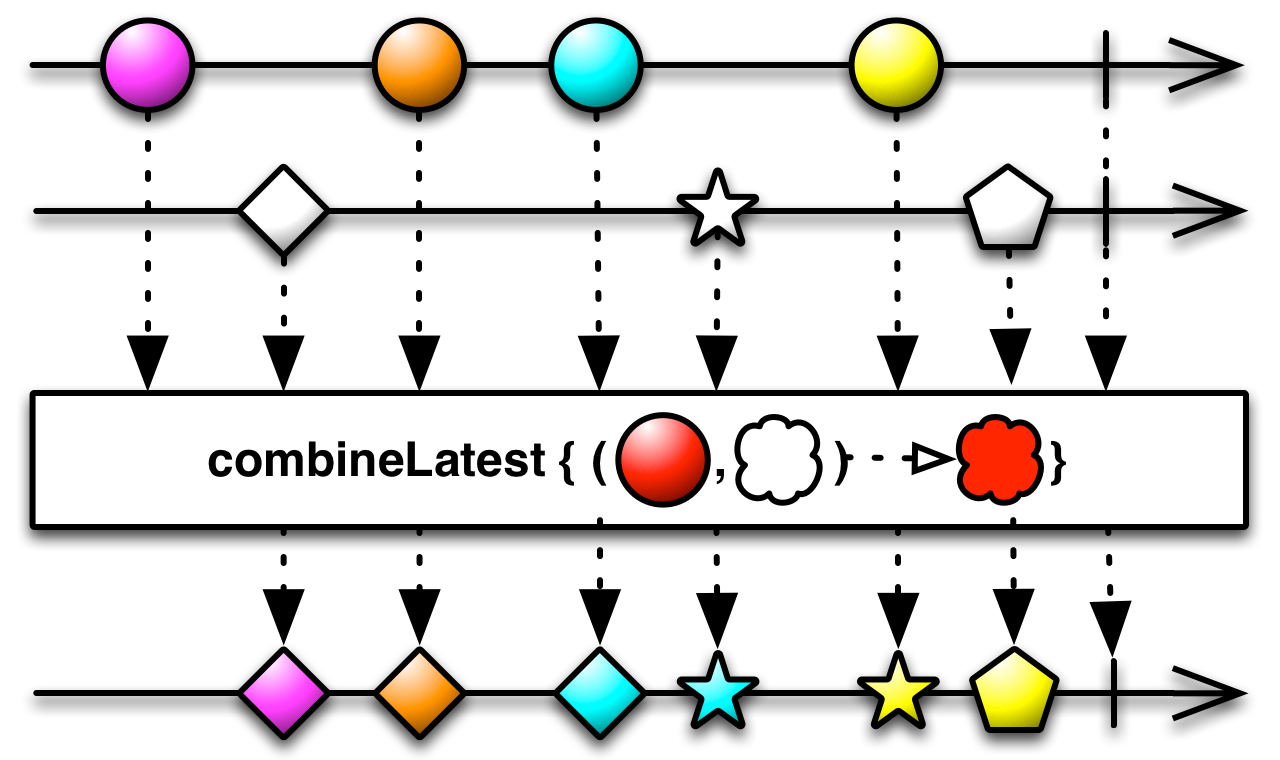### #combineLatestSource

``combineLatest :: forall f c b a. Apply f => (a -> b -> c) -> ObservableT f a -> ObservableT f b -> ObservableT f c``

An Observable of projected values from the most recent values from each input### #mergeSource

``merge :: forall f a. Apply f => ObservableT f a -> ObservableT f a -> ObservableT f a``

Creates an output ObservableImpl which concurrently emits all values from each input ObservableImpl.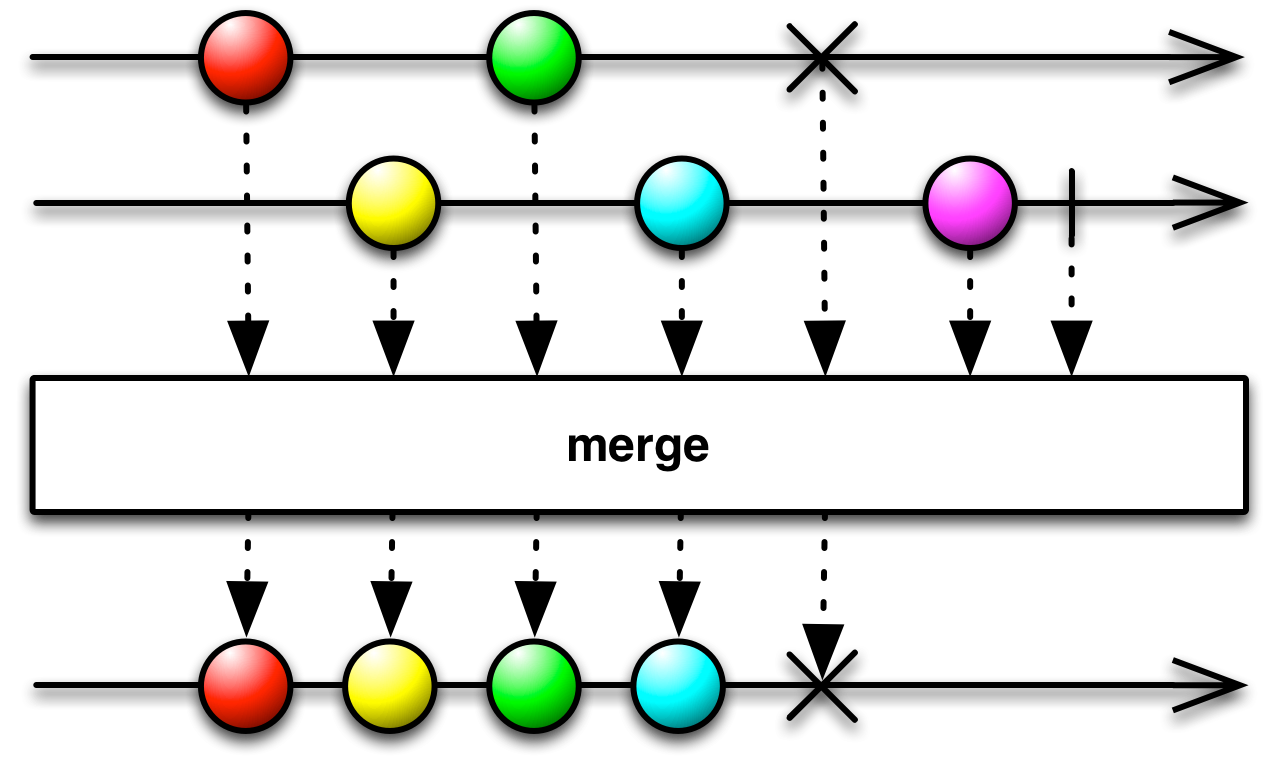### #throwSource

``throw :: forall f a. Applicative f => Error -> ObservableT f a``

Creates an ObservableImpl that immediately sends an error notification.

### #justSource

``just :: forall f a. Applicative f => a -> ObservableT f a``

Creates an ObservableImpl that emits the value specify, and then emits a complete notification. An alias for `of`.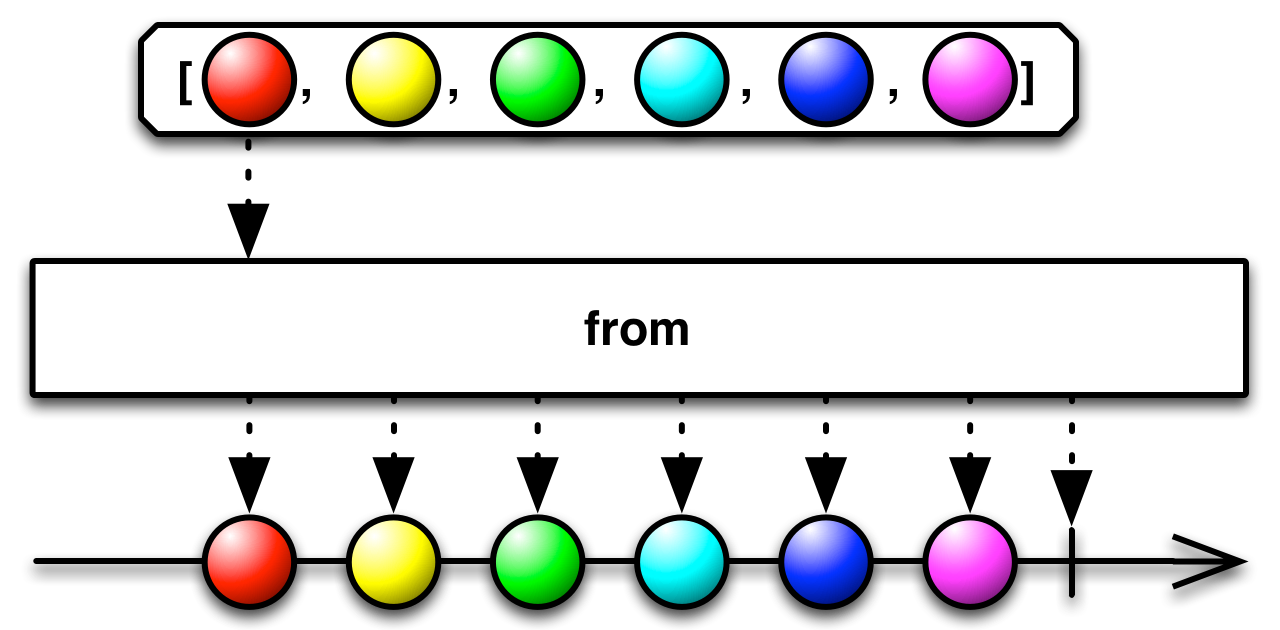### #ajaxUrlSource

``ajaxUrl :: forall e. String -> ObservableT (Eff e) Response``

### #ajaxSource

``ajax :: forall e. Request -> ObservableT (Eff e) Response``

### #neverSource

``never :: forall f a. Applicative f => ObservableT f a``

Creates an ObservableImpl that emits no items. Subscriptions it must be disposed manually.### #fromArraySource

``fromArray :: forall f a. Applicative f => Array a -> ObservableT f a``

Creates an ObservableImpl from an Array.### #fromEventSource

``fromEvent :: forall e. EventTarget -> EventType -> ObservableT (Eff (dom :: DOM | e)) Event``

Creates an ObservableImpl that emits events of the specified type coming from the given event target.

### #intervalSource

``interval :: forall f. Applicative f => Int -> ObservableT f Int``

Returns an ObservableImpl that emits an infinite sequence of ascending integers, with a constant interval of time of your choosing between those emissions.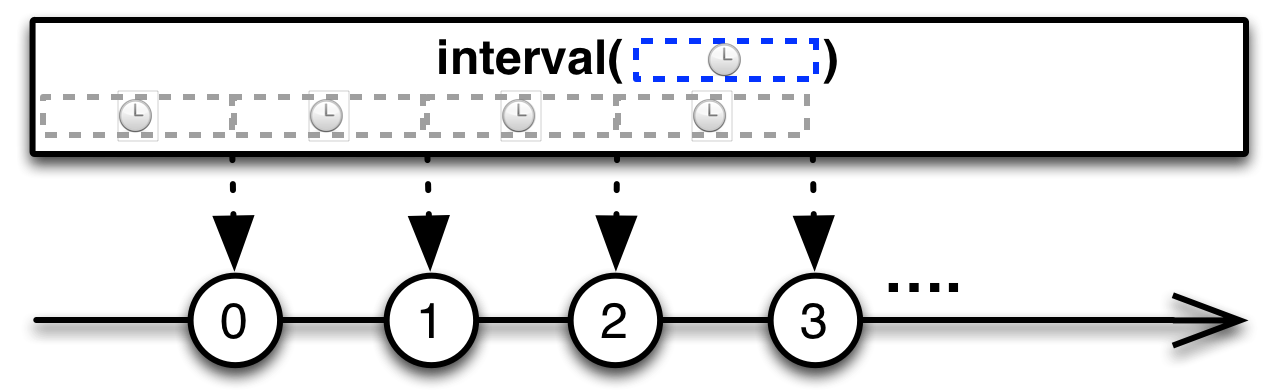### #rangeSource

``range :: forall f. Applicative f => Int -> Int -> ObservableT f Int``

The range operator emits a range of sequential integers, in order, where you select the start of the range and its length### #timerSource

``timer :: forall f. Applicative f => Int -> Int -> ObservableT f Int``

Creates an Observable that, upon subscription, emits and infinite sequence of ascending integers, after a specified delay, every specified period. Delay and period are in milliseconds.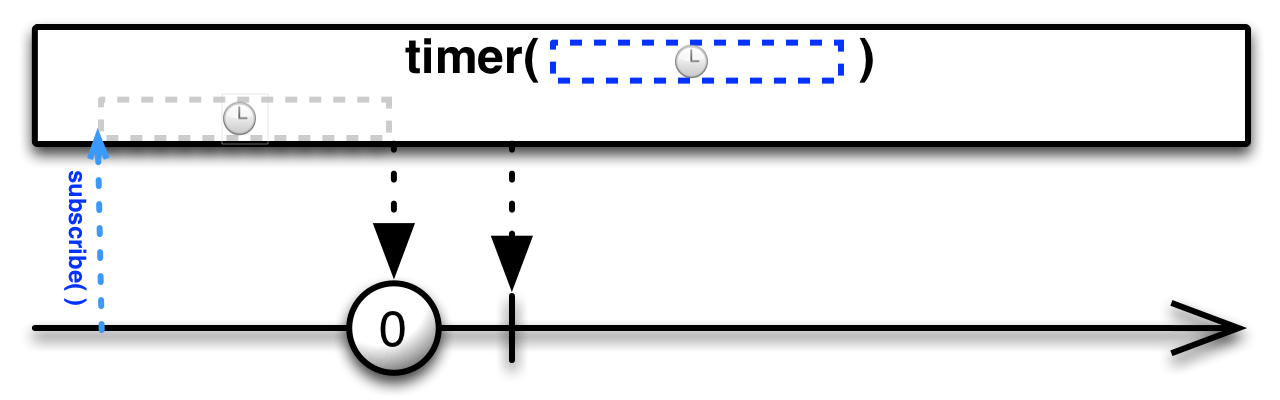### #createSource

``create :: forall u e a. (Subscriber a -> Eff e u) -> ObservableT (Eff e) a``

### #bufferSource

``buffer :: forall f b a. Apply f => ObservableT f b -> ObservableT f a -> ObservableT f (Array a)``

Collects values from the first Observable into an Array, and emits that array only when second Observable emits.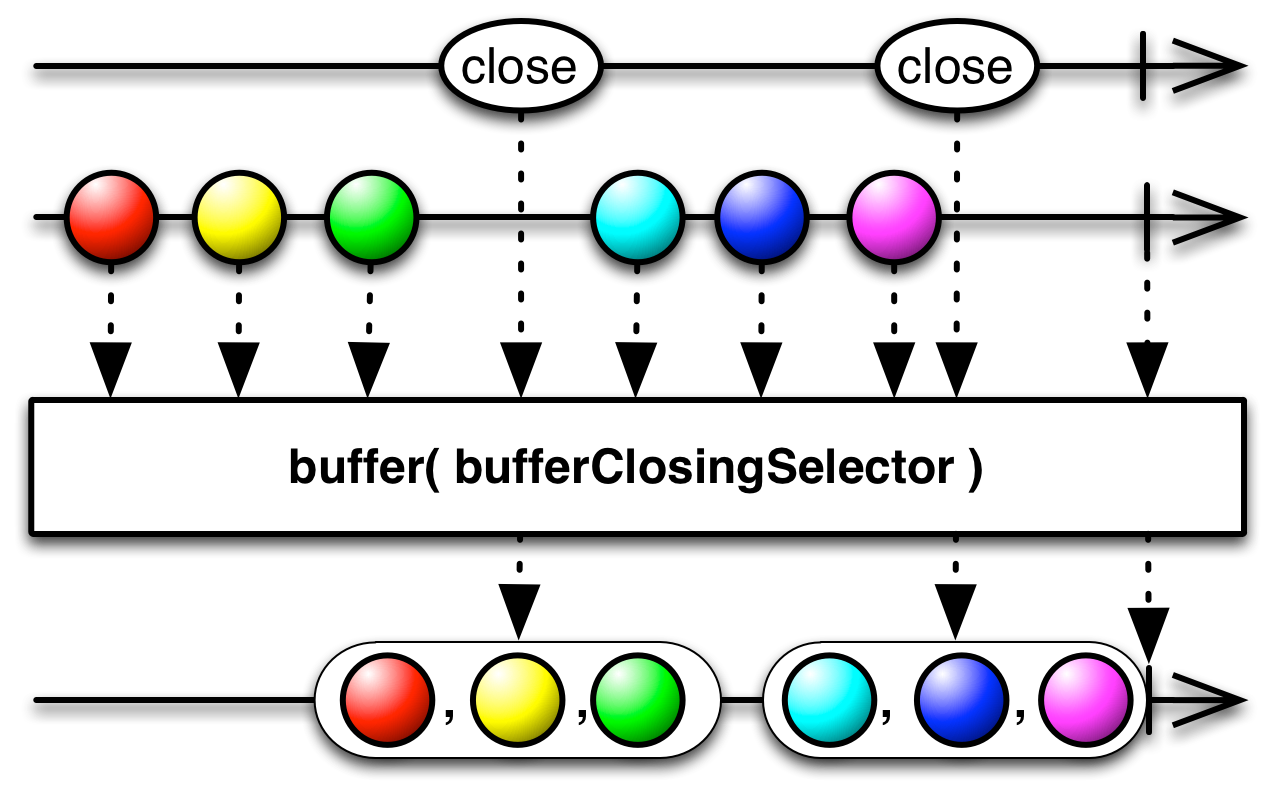### #bufferCountSource

``bufferCount :: forall f a. Functor f => Int -> Int -> ObservableT f a -> ObservableT f (Array a)``

Collects values from the past as an array, emits that array when its size (arg1) reaches the specified buffer size, and starts a new buffer. The new buffer starts with nth (arg2) element of the Observable counting from the beginning of the last buffer.### #bufferTimeSource

``bufferTime :: forall f a. Functor f => Int -> Int -> Int -> (ObservableT f a) -> (ObservableT f (Array a))``

Collects values from the past as an array, and emits those arrays periodically in time. The first argument is how long to fill the buffer. The second argument is specifies when to open the next buffer following an emission. The third argument is the maximum size of any buffer.

### #mapToSource

``mapTo :: forall f b a. Functor f => b -> ObservableT f a -> ObservableT f b``

Emits the given constant value on the output Observable every time the source Observable emits a value.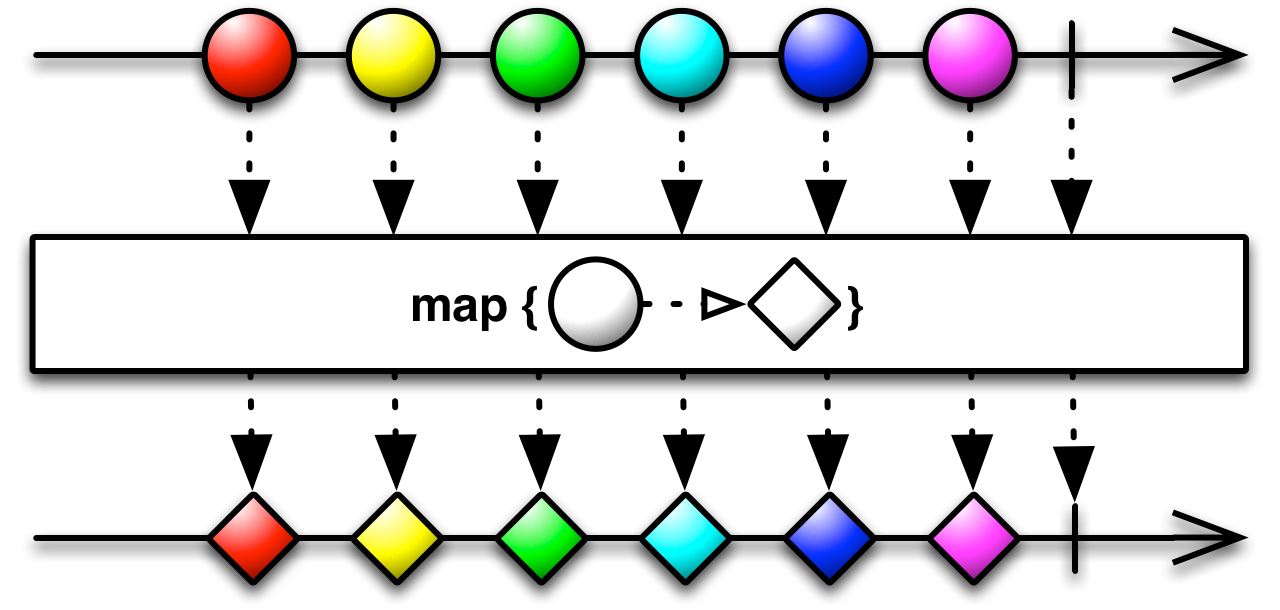### #pairwiseSource

``pairwise :: forall f a. Functor f => ObservableT f a -> ObservableT f (Tuple a a)``

Puts the current value and previous value together as an array, and emits that.### #partitionSource

``partition :: forall f a. Applicative f => (a -> Boolean) -> ObservableT f a -> Tuple (ObservableT f a) (ObservableT f a)``

Given a predicate function (arg1), and an Observable (arg2), it outputs a two element array of partitioned values (i.e., [ Observable valuesThatPassPredicate, Observable valuesThatFailPredicate ]).### #mergeMapSource

``mergeMap :: forall m b a. Monad m => ObservableT m a -> (a -> ObservableT m b) -> ObservableT m b``

Maps each value to an Observable, then flattens all of these Observables using mergeAll. It's just monadic `bind`.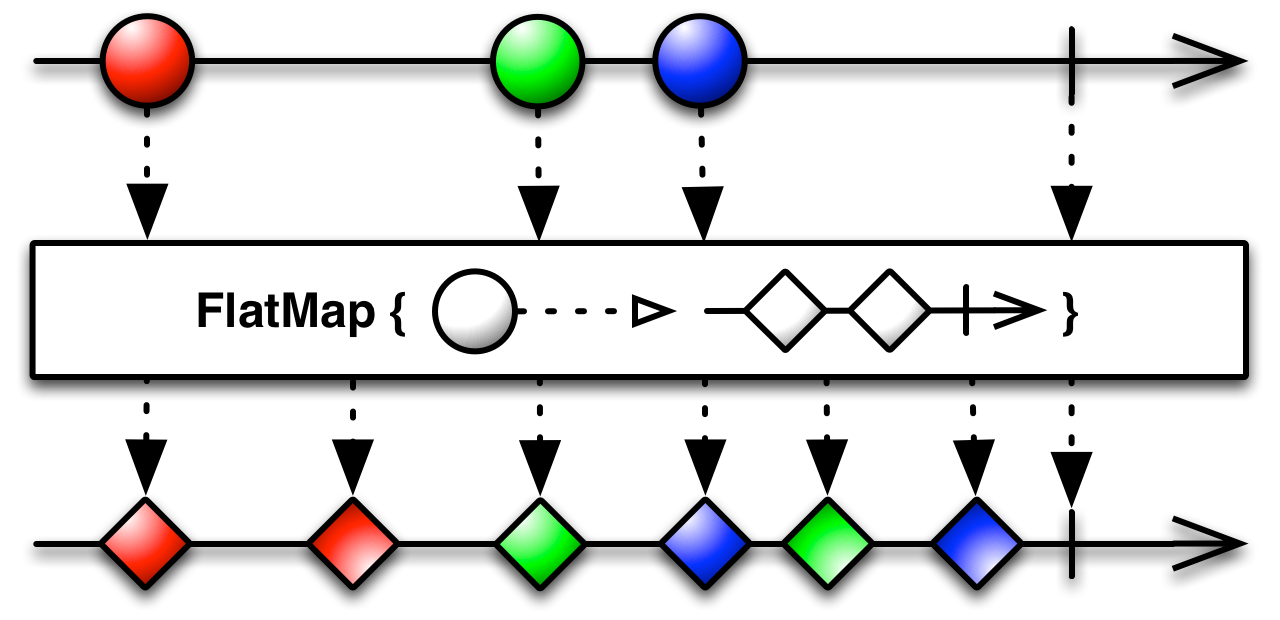### #mergeMapToSource

``mergeMapTo :: forall m b a. Apply m => ObservableT m a -> ObservableT m b -> ObservableT m b``

Maps each value of the ObservableImpl (arg1) to the same inner ObservableImpl (arg2), then flattens the result.### #scanSource

``scan :: forall f b a. Functor f => (a -> b -> b) -> b -> ObservableT f a -> ObservableT f b``

Given an accumulator function (arg1), an initial value (arg2), and a source ObservableImpl (arg3), it returns an ObservableImpl that emits the current accumlation whenever the source emits a value.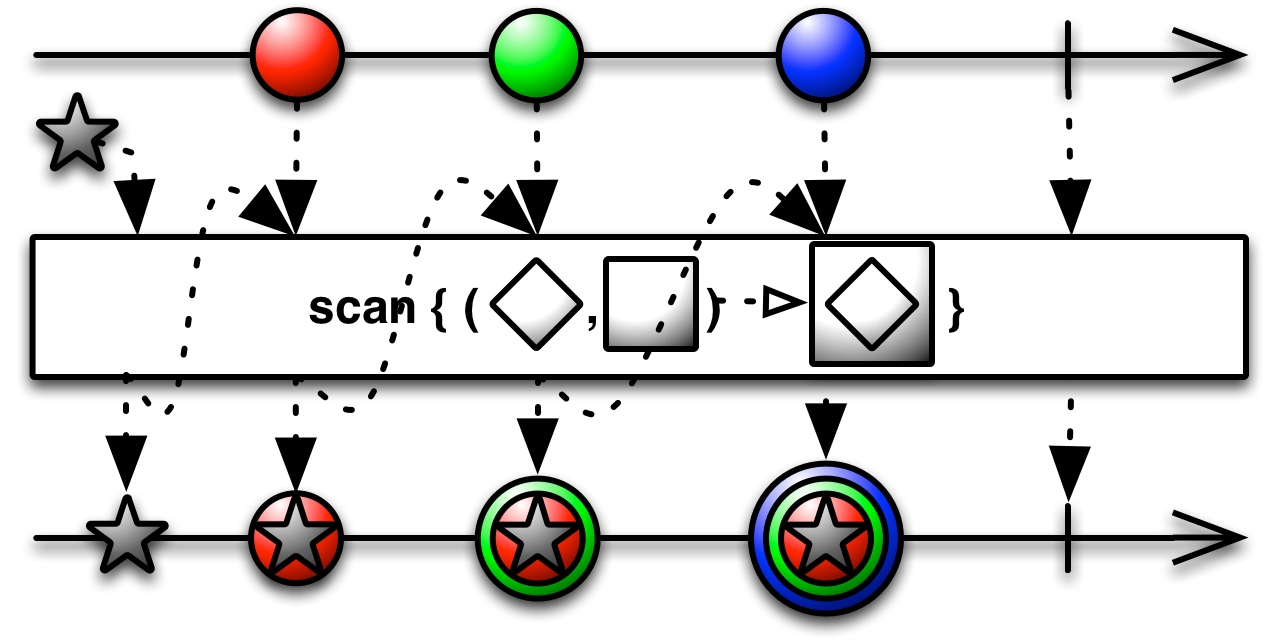### #debounceTimeSource

``debounceTime :: forall f a. Functor f => Int -> ObservableT f a -> ObservableT f a``

It's like delay, but passes only the most recent value from each burst of emissions.

### #distinctSource

``distinct :: forall f a. Functor f => ObservableT f a -> ObservableT f a``

Returns an Observable that emits all items emitted by the source Observable that are distinct by comparison from previous items.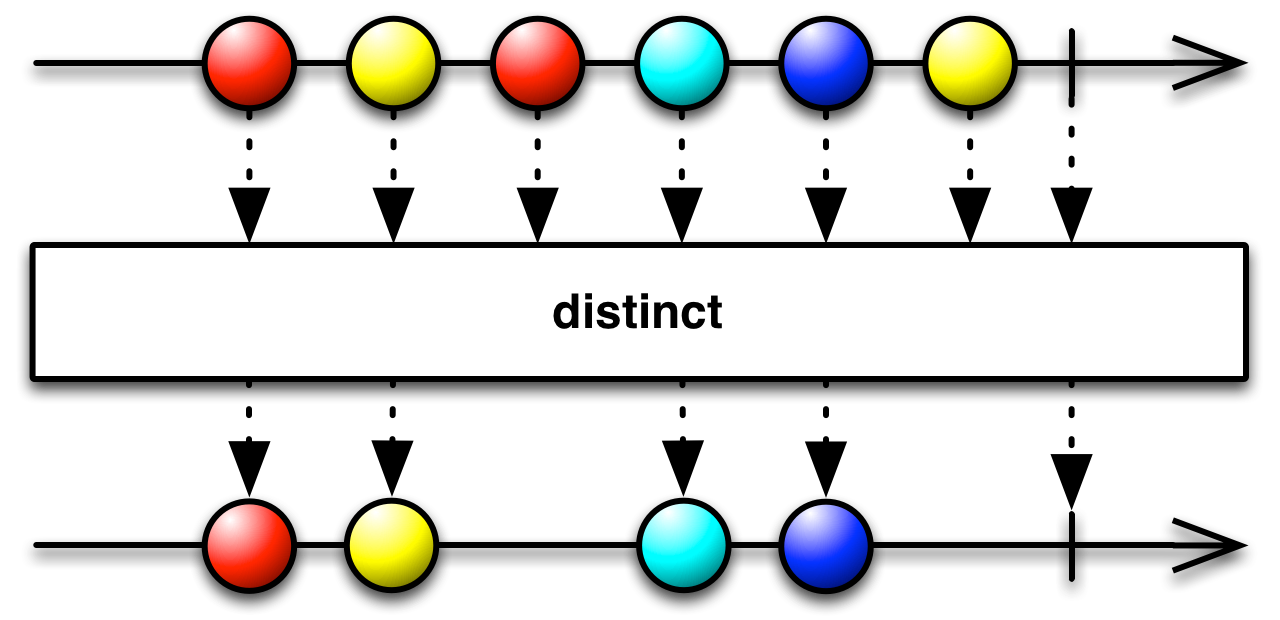### #distinctUntilChangedSource

``distinctUntilChanged :: forall f a. Functor f => ObservableT f a -> ObservableT f a``

Returns an Observable that emits all items emitted by the source Observable that are distinct by comparison from the previous item.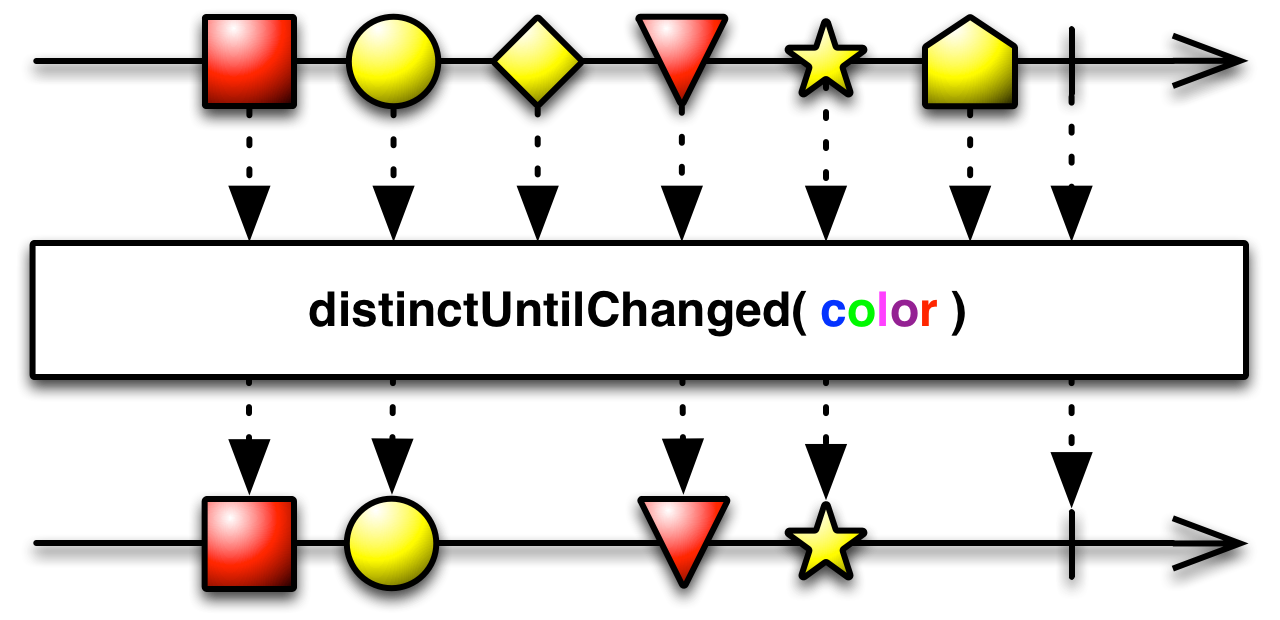### #elementAtSource

``elementAt :: forall f a. Functor f => Int -> ObservableT f a -> ObservableT f a``

Emits the single value at the specified index in a sequence of emissions from the source

### #filterSource

``filter :: forall f a. Functor f => (a -> Boolean) -> ObservableT f a -> ObservableT f a``

Filter items emitted by the source Observable by only emitting those that satisfy a specified predicate.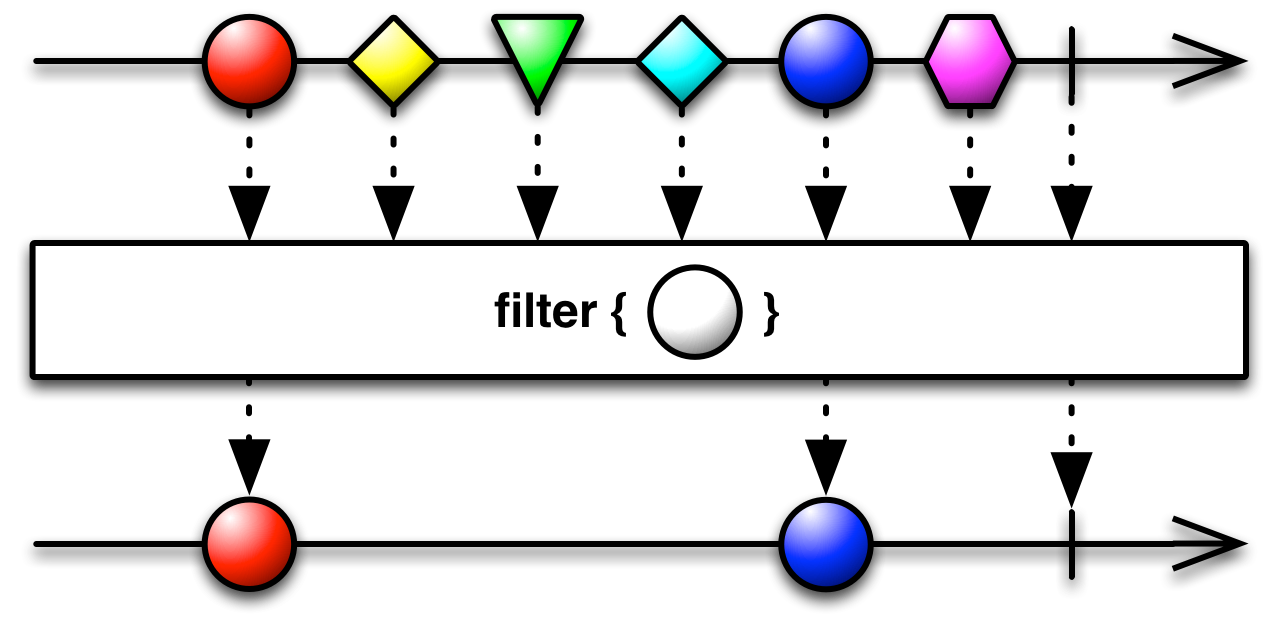### #ignoreElementsSource

``ignoreElements :: forall f a. Functor f => ObservableT f a -> ObservableT f a``

Ignores all items emitted by the source Observable and only passes calls of complete or error.### #lastSource

``last :: forall f a. Functor f => (a -> Boolean) -> ObservableT f a -> ObservableT f a``

Returns an Observable that emits only the last item emitted by the source Observable that that satisfies the given predicate.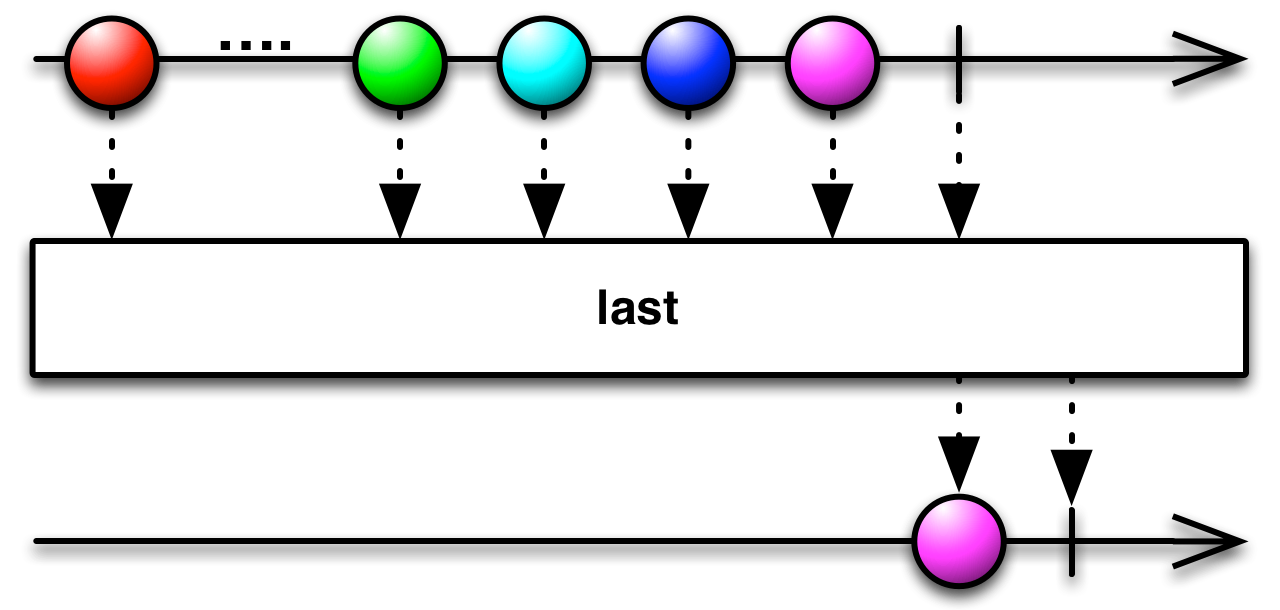### #sampleSource

``sample :: forall f b a. Apply f => ObservableT f b -> ObservableT f a -> ObservableT f a``

It's like sampleTime, but samples whenever the notifier Observable emits something.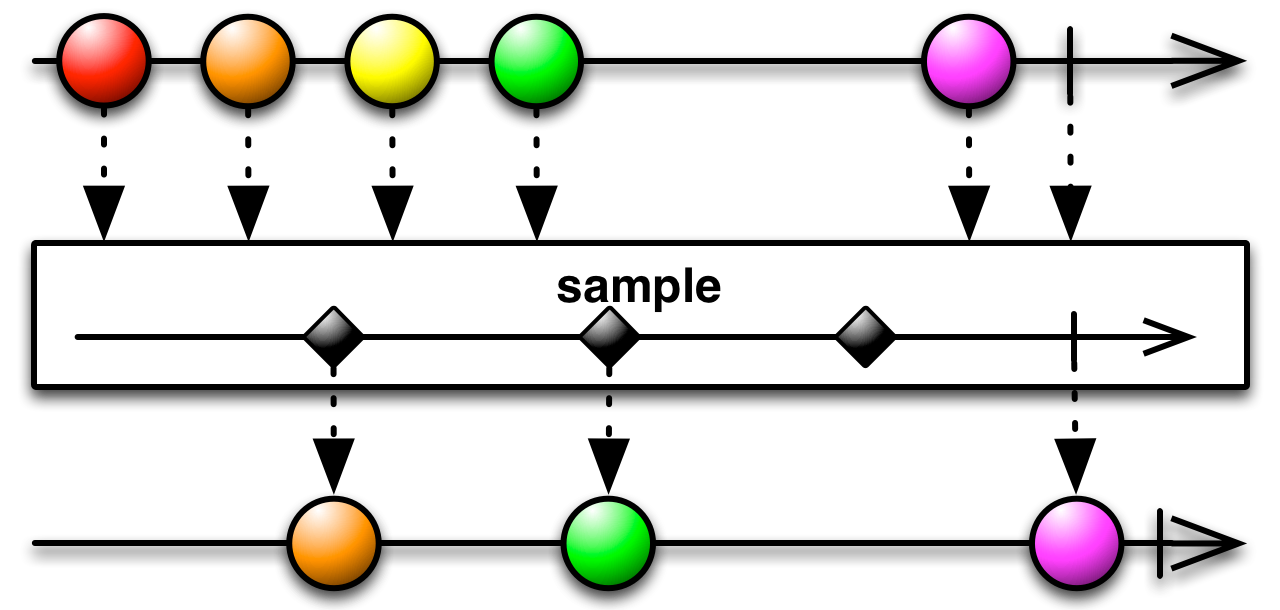### #sampleTimeSource

``sampleTime :: forall f a. Functor f => Int -> ObservableT f a -> ObservableT f a``

Periodically looks at the source Observable and emits whichever value it has most recently emitted since the previous sampling, unless the source has not emitted anything since the previous sampling.

### #skipSource

``skip :: forall f a. Functor f => Int -> ObservableT f a -> ObservableT f a``

Returns an Observable that skips n items emitted by an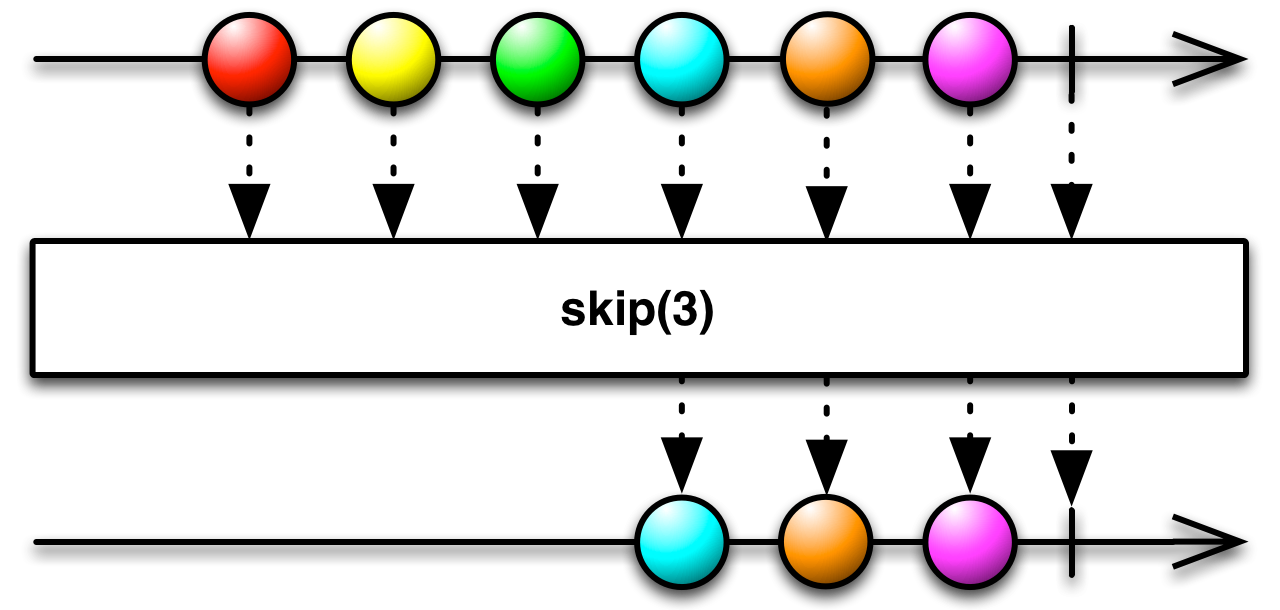### #skipUntilSource

``skipUntil :: forall f b a. Apply f => ObservableT f b -> ObservableT f a -> ObservableT f a``

Returns an Observable that skips items emitted by the source Observable until a second Observable emits an item.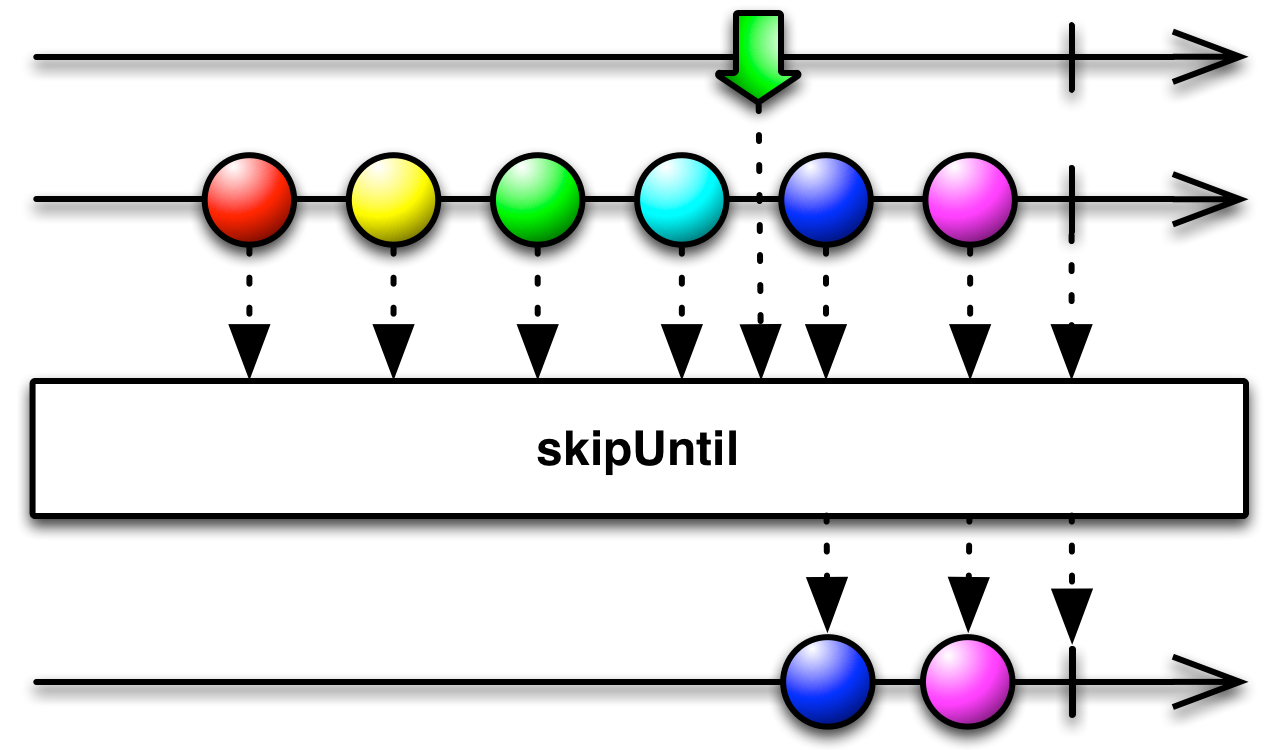### #skipWhileSource

``skipWhile :: forall f a. Functor f => (a -> Boolean) -> ObservableT f a -> ObservableT f a``

Returns an Observable that skips all items emitted by the source Observable as long as a specified condition holds true, but emits all further source items as soon as the condition becomes false.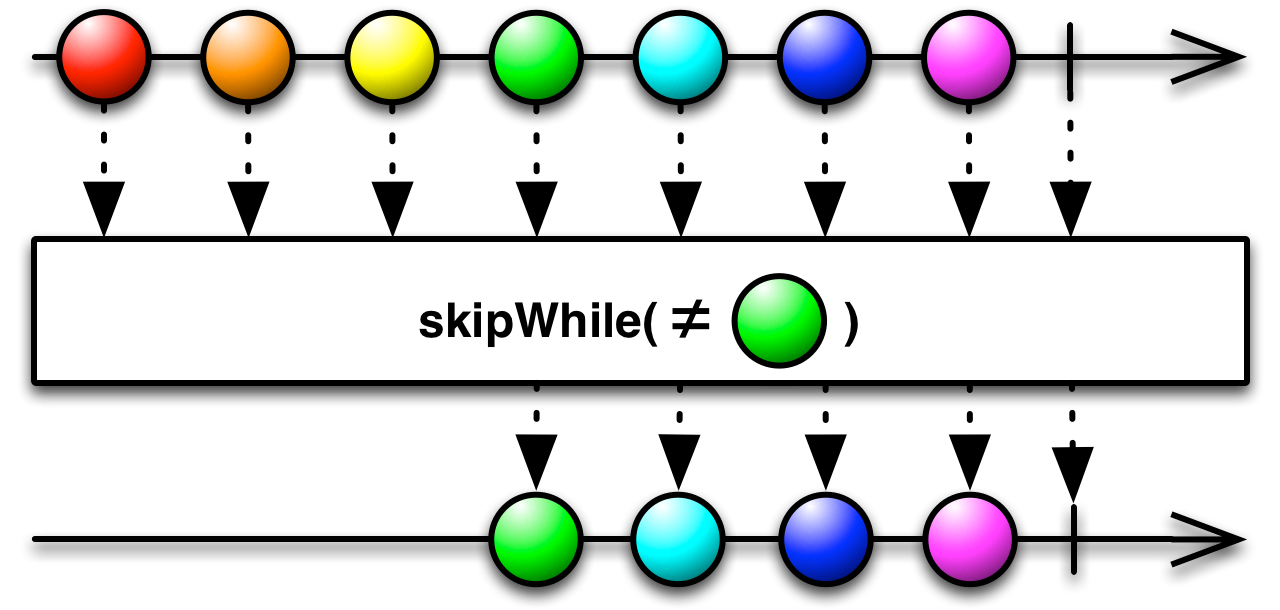### #takeSource

``take :: forall f a. Functor f => Int -> ObservableT f a -> ObservableT f a``

Emits only the first n values emitted by the source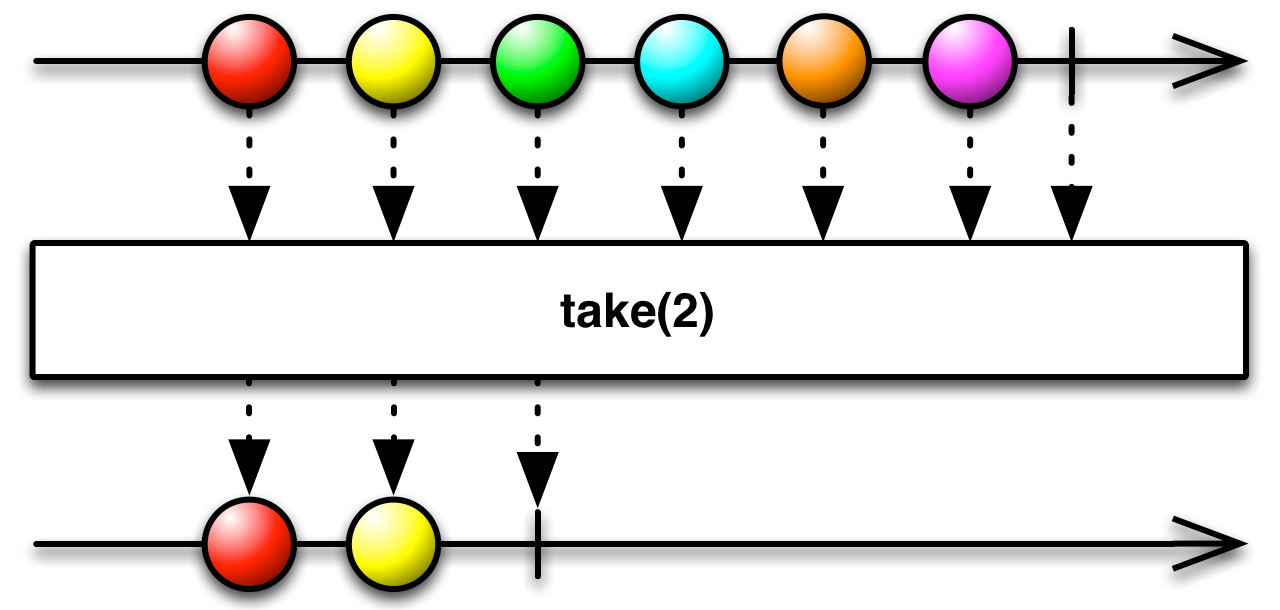### #takeUntilSource

``takeUntil :: forall f b a. Apply f => ObservableT f b -> ObservableT f a -> ObservableT f a``

Lets values pass until a second Observable emits something. Then, it completes. ![marble diagram](https://raw.githubusercontent.com/wiki/ReactiveX/RxJava/images/rx-operators/takeUntil.png" alt=""

### #takeWhileSource

``takeWhile :: forall f a. Functor f => (a -> Boolean) -> ObservableT f a -> ObservableT f a``

Emits values emitted by the source Observable so long as each value satisfies the given predicate, and then completes as soon as this predicate is not satisfied.

### #auditTimeSource

``auditTime :: forall f a. Functor f => Int -> ObservableT f a -> ObservableT f a``

Ignores source values for duration milliseconds, then emits the most recent value from the source Observable, then repeats this process.### #throttleTimeSource

``throttleTime :: forall f a. Functor f => Int -> ObservableT f a -> ObservableT f a``

Emits a value from the source Observable, then ignores subsequent source values for duration milliseconds, then repeats this process.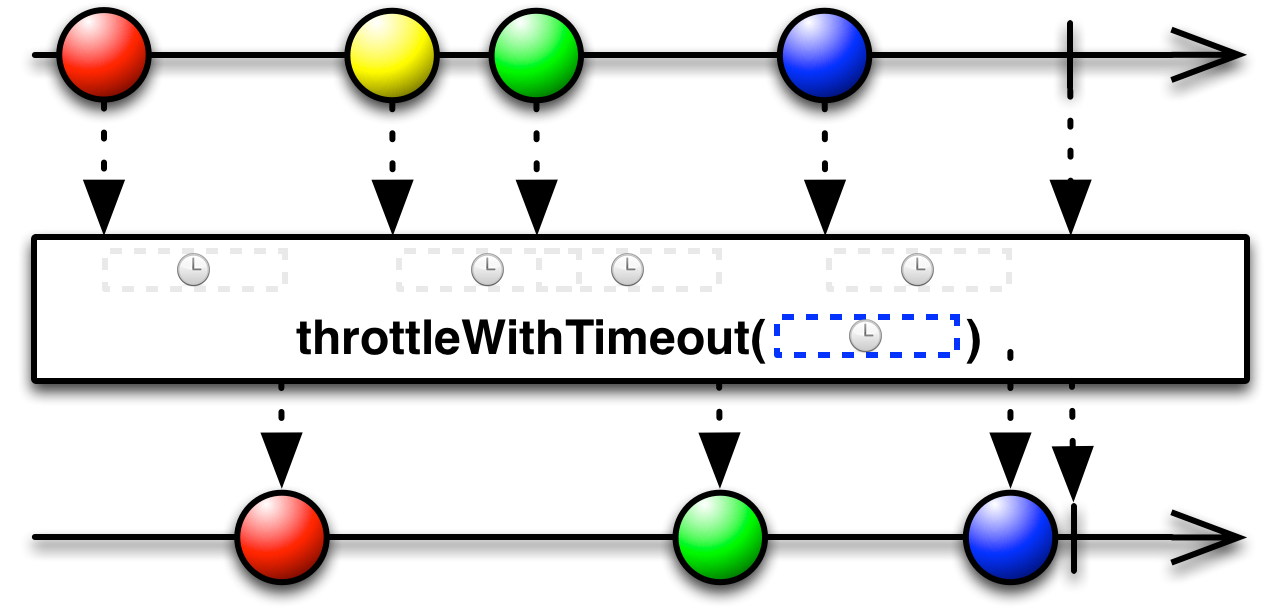### #startWithManySource

``startWithMany :: forall m a f. Foldable f => Functor m => f a -> ObservableT m a -> ObservableT m a``

Returns an Observable that emits the items in the given Foldable before it begins to emit items emitted by the source Observable.

### #startWithSource

``startWith :: forall f a. Functor f => a -> ObservableT f a -> ObservableT f a``

Returns an Observable that emits the item given before it begins to emit items emitted by the source Observable.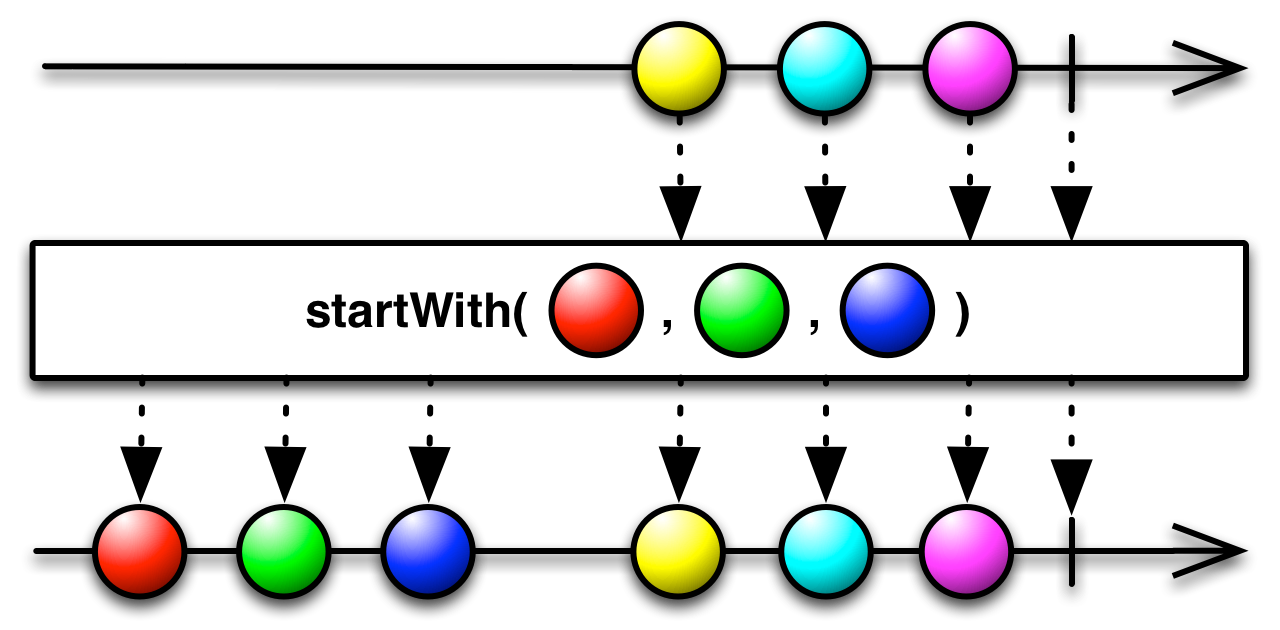### #withLatestFromSource

``withLatestFrom :: forall f c b a. Apply f => (a -> b -> c) -> ObservableT f b -> ObservableT f a -> ObservableT f c``

Combines each value from the source Observables using a project function to determine the value to be emitted on the output ![marble diagram](https://raw.github.com/wiki/ReactiveX/RxJava/images/rx-operators/withLatestFrom.png" alt="">

### #concatSource

``concat :: forall f a. Apply f => ObservableT f a -> ObservableT f a -> ObservableT f a``

Concatenates two Observables together by sequentially emitting their values, one Observable after the other.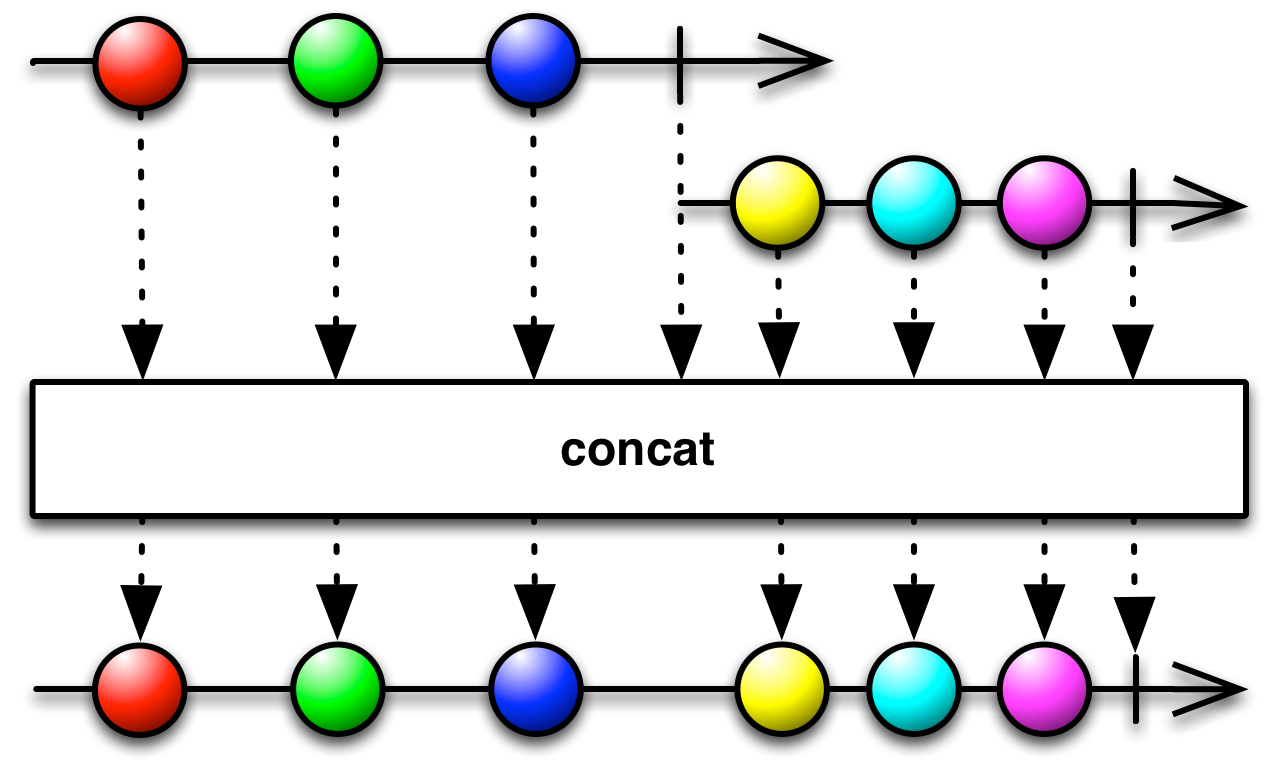### #switchMapToSource

``switchMapTo :: forall f b a. Apply f => ObservableT f b -> ObservableT f a -> ObservableT f b``

It's like switchMap, but maps each value to the same inner ObservableImpl.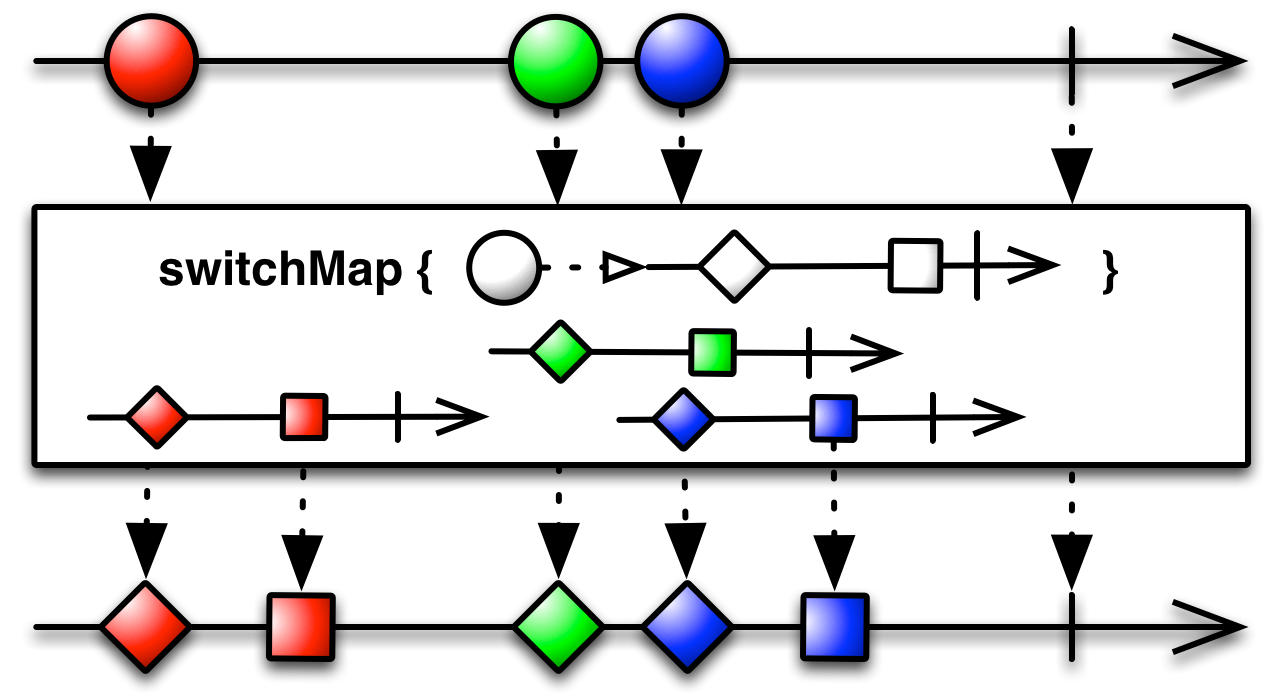### #retrySource

``retry :: forall f a. Functor f => Int -> ObservableT f a -> ObservableT f a``

If the source Observable calls error, this method will resubscribe to the source Observable n times rather than propagating the error call.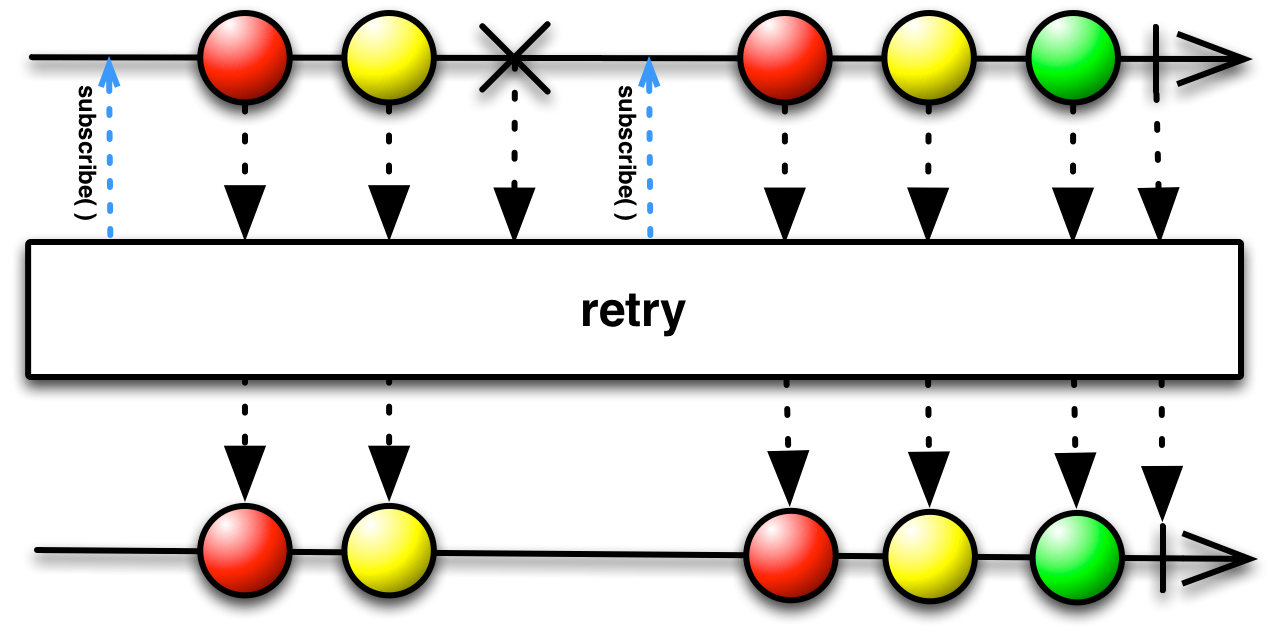### #delaySource

``delay :: forall f a. Functor f => Int -> ObservableT f a -> ObservableT f a``

Time shifts each item by some specified amount of milliseconds.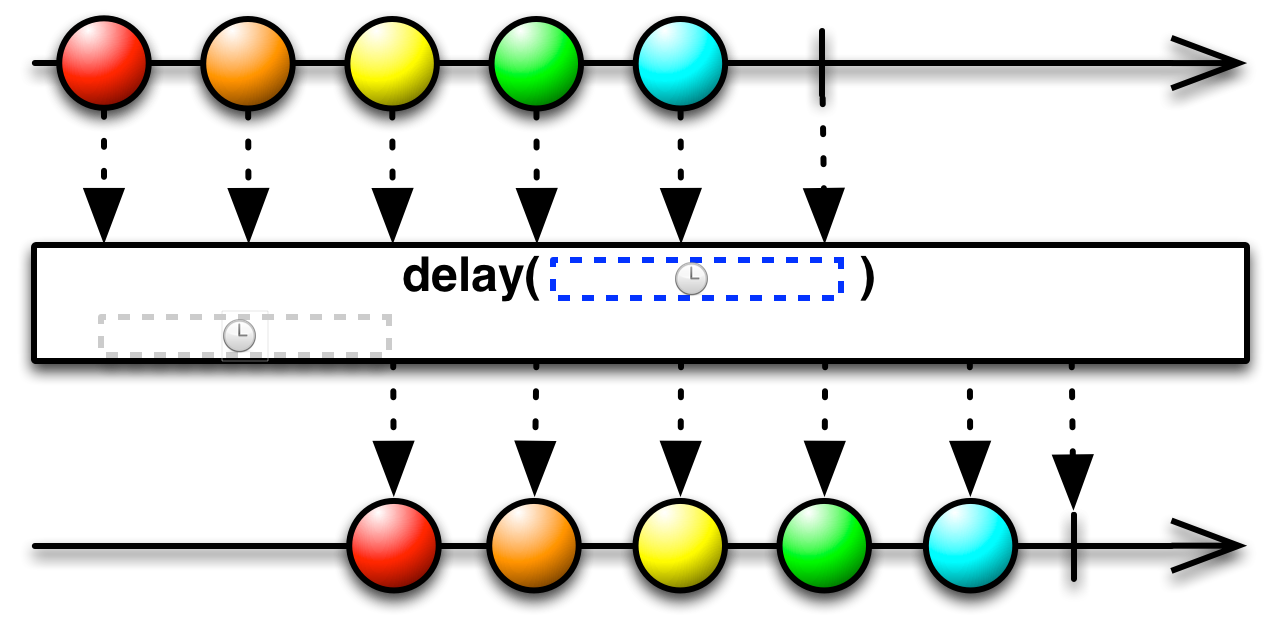### #defaultIfEmptySource

``defaultIfEmpty :: forall f a. Functor f => a -> ObservableT f a -> ObservableT f a``

Returns an Observable that emits the items emitted by the source Observable or a specified default item if the source Observable is empty.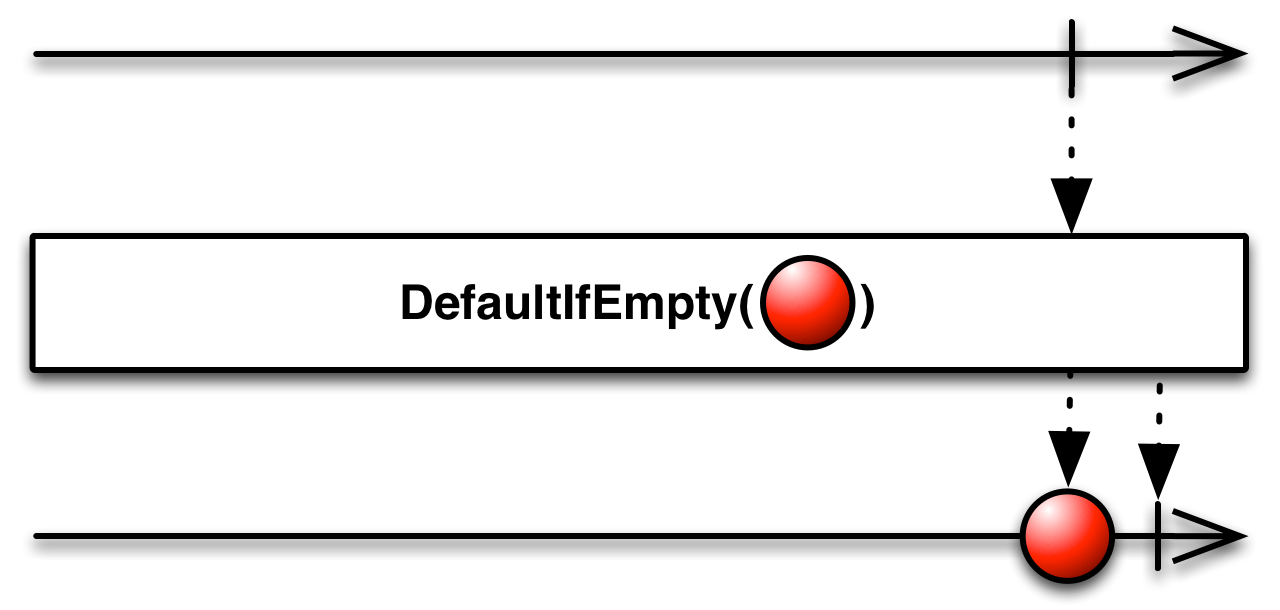takes a defaultValue which is the item to emit if the source Observable emits no items.

returns an Observable that emits either the specified default item if the source Observable emits no items, or the items emitted by the source Observable

### #everySource

``every :: forall f a. Functor f => (a -> Boolean) -> ObservableT f a -> ObservableT f Boolean``

Determines whether all elements of an observable sequence satisfy a condition. Returns an observable sequence containing a single element determining whether all elements in the source sequence pass the test in the specified predicate.

### #isEmptySource

``isEmpty :: forall f a. Functor f => ObservableT f a -> ObservableT f Boolean``

Tests whether this `Observable` emits no elements.

returns an Observable emitting one single Boolean, which is `true` if this `Observable` emits no elements, and `false` otherwise.

### #shareSource

``share :: forall f a. Functor f => ObservableT f a -> ObservableT f a``

Returns a new Observable that multicasts (shares) the original Observable. As long a there is more than 1 Subscriber, this Observable will be subscribed and emitting data. When all subscribers have unsubscribed it will unsubscribe from the source Observable.

This is an alias for `publish().refCount()`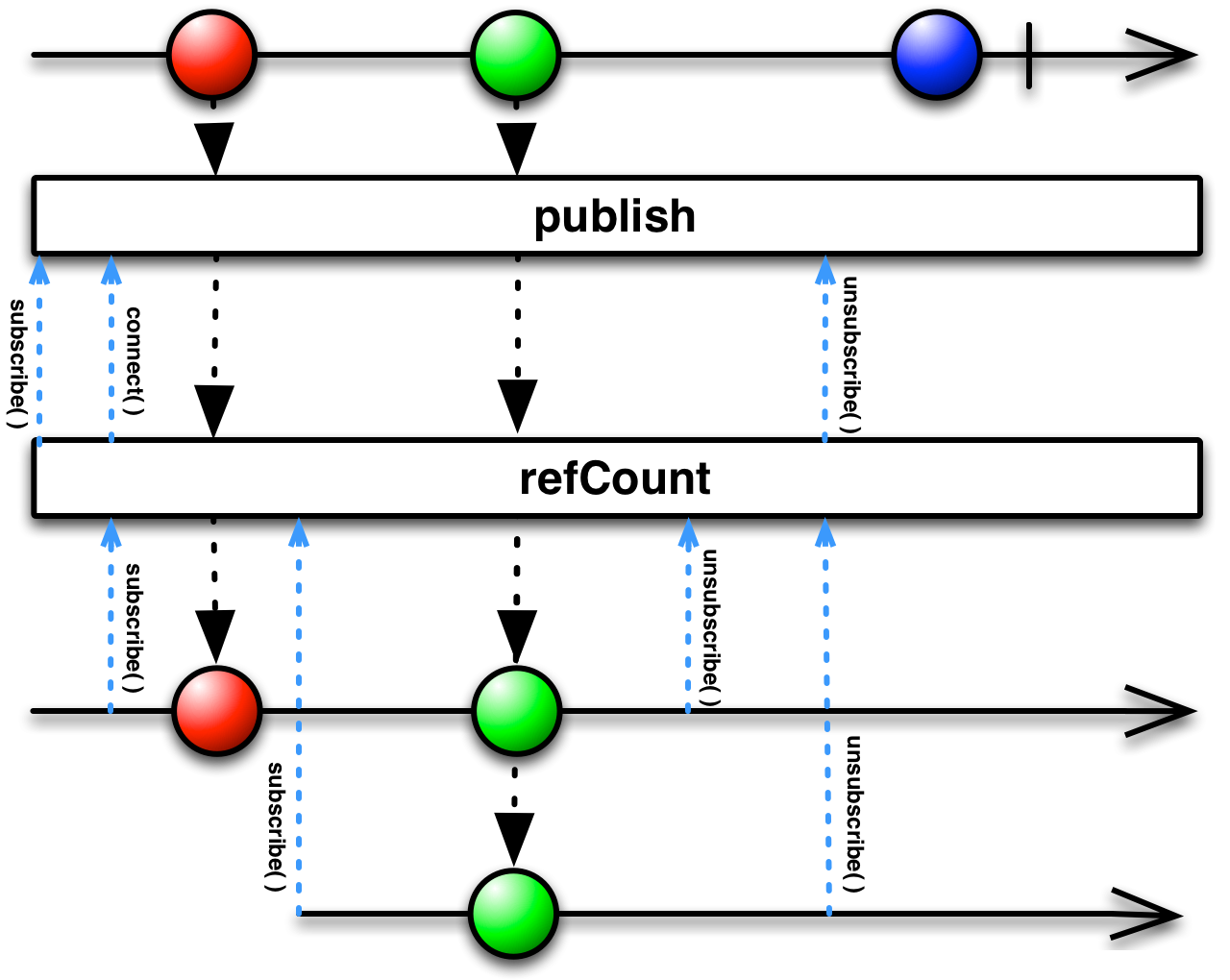returns an Observable that upon connection causes the source Observable to emit items to its Subscribers

### #firstSource

``first :: forall f a. Functor f => (a -> Boolean) -> ObservableT f a -> ObservableT f a``

Returns an Observable that emits only the first item emitted by the source Observable that satisfies the given predicate.

### #countSource

``count :: forall f a. Functor f => ObservableT f a -> ObservableT f Int``

Counts the number of emissions on the source and emits that number when the source completes.

### #reduceSource

``reduce :: forall f b a. Functor f => (a -> b -> b) -> b -> ObservableT f a -> ObservableT f b``

Applies an accumulator function over the source Observable, and returns the accumulated result when the source completes, given a seed value.

### #observeOnSource

``observeOn :: forall f a. Functor f => Scheduler -> ObservableT f a -> ObservableT f a``

Makes every `next` call run in the new Scheduler.

### #subscribeOnSource

``subscribeOn :: forall f a. Functor f => Scheduler -> ObservableT f a -> ObservableT f a``

Makes subscription happen on a given Scheduler.

### #subscribeNextSource

``subscribeNext :: forall u e f a. Functor f => (a -> Eff e u) -> ObservableT f a -> f (Eff e Subscription)``

### #subscribeSource

``subscribe :: forall e f a. Functor f => Subscriber a -> ObservableT f a -> f (Eff e Subscription)``

Subscribing to an ObservableImpl is like calling a function, providing `next`, `error` and `completed` effects to which the data will be delivered.

### #dematerializeSource

``dematerialize :: forall f a. Functor f => ObservableT f (Notification a) -> ObservableT f a``

Returns an ObservableImpl that reverses the effect of `materialize` by `Notification` objects emitted by the source ObservableImpl into the items or notifications they represent.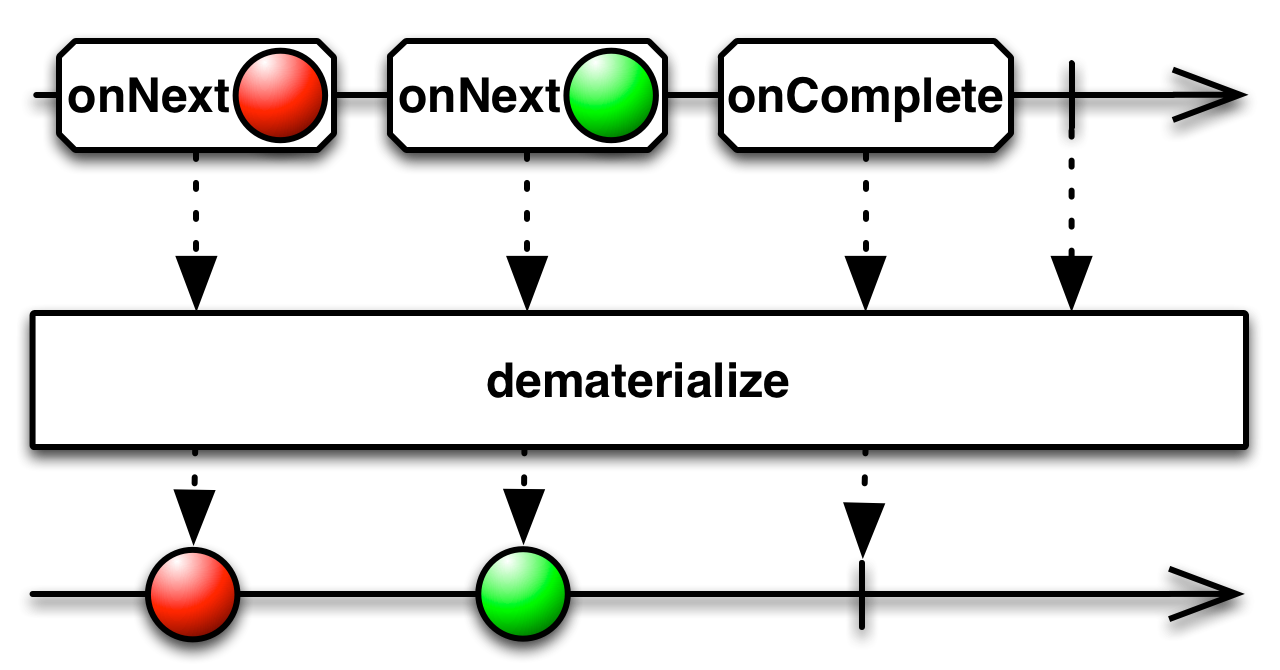### #materializeSource

``materialize :: forall f a. Functor f => ObservableT f a -> ObservableT f (Notification a)``

Turns all of the notifications from a source ObservableImpl into onNext emissions, and marks them with their original notification types within `Notification` objects.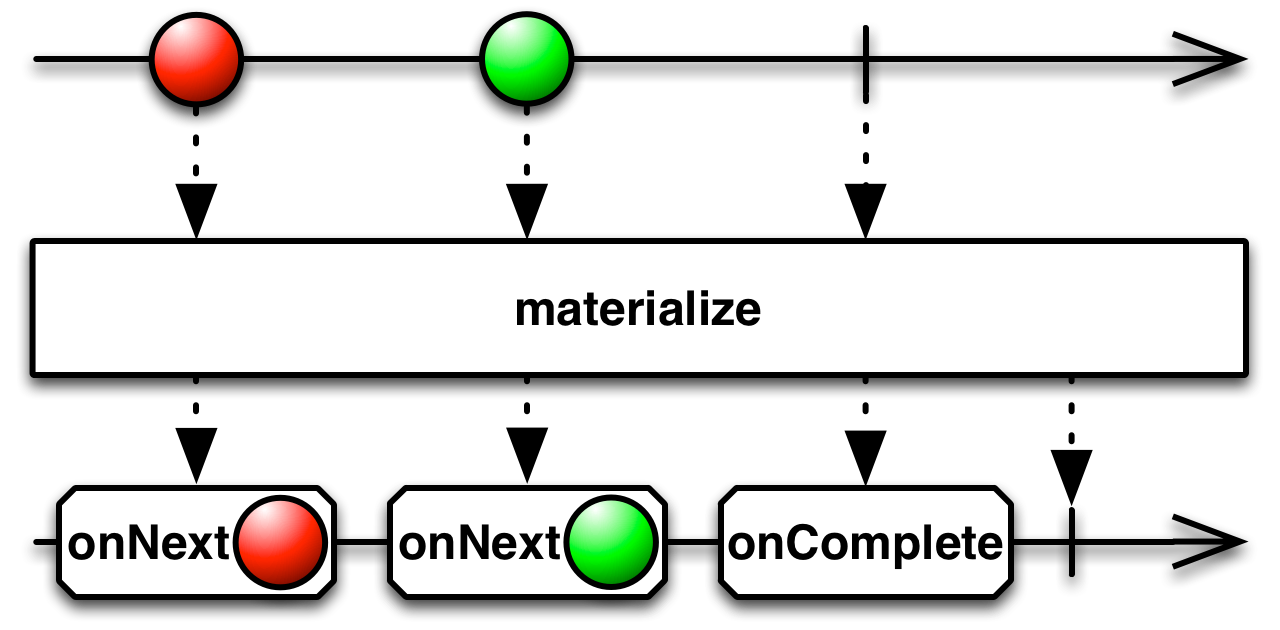### #toArraySource

``toArray :: forall f a. Functor f => ObservableT f a -> ObservableT f (Array a)``

Returns an ObservableImpl that emits a single item, a list composed of all the items emitted by the source ObservableImpl.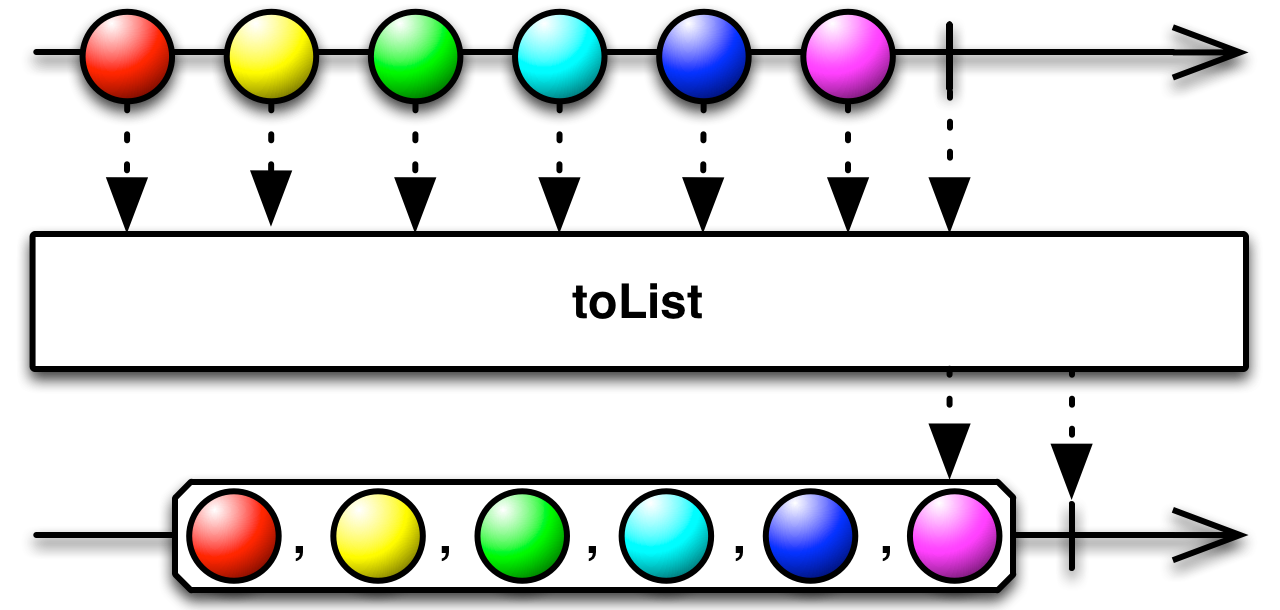### #auditSource

``audit :: forall b a. (a -> Observable b) -> Observable a -> Observable a``

It's like auditTime, but the silencing duration is determined by a second ObservableImpl.### #debounceSource

``debounce :: forall a. (a -> Observable Int) -> Observable a -> Observable a``

It's like debounceTime, but the time span of emission silence is determined by a second ObservableImpl. Allows for a variable debounce rate.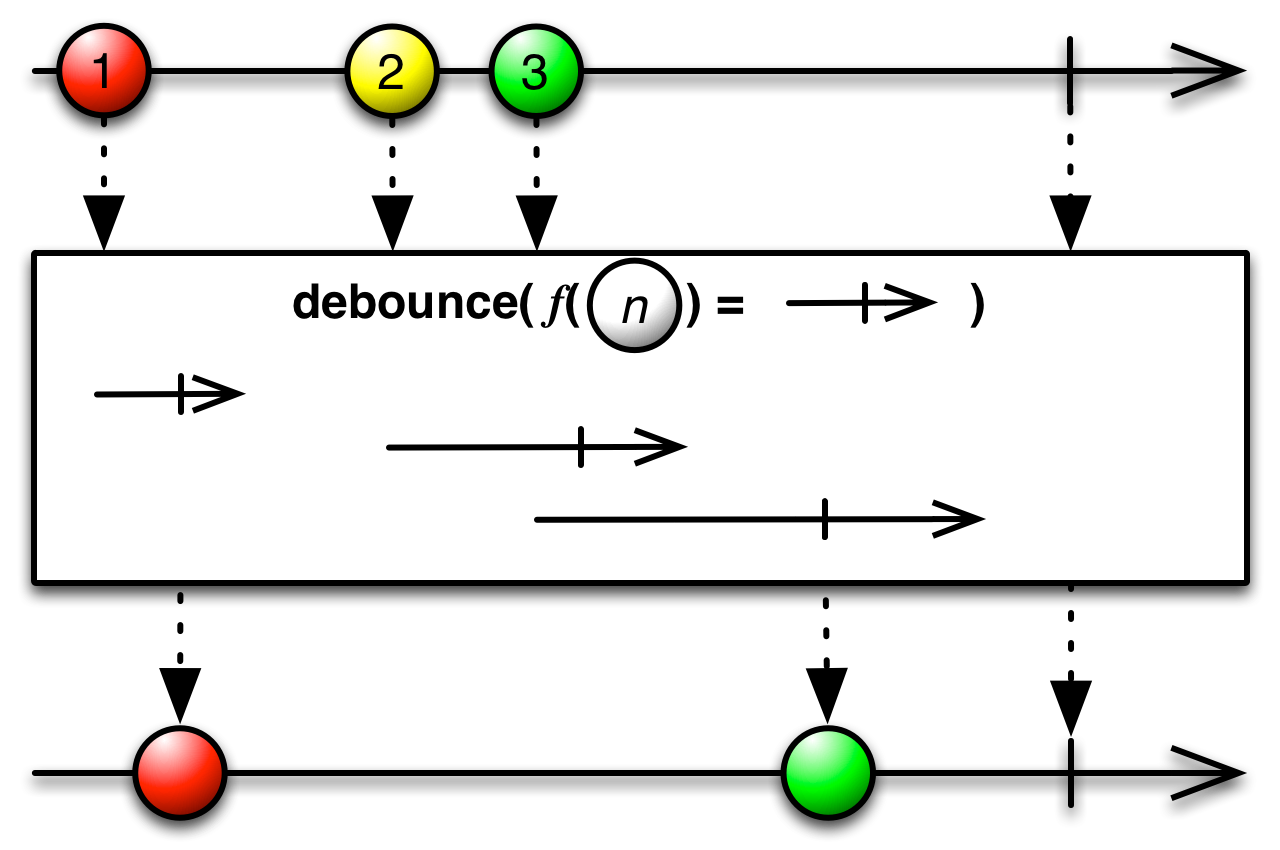### #bufferWhenSource

``bufferWhen :: forall b a. (a -> Observable b) -> Observable a -> Observable (Array a)``

Collects values from the past as an array. When it starts collecting values, it calls a function that returns an ObservableImpl that emits to close the buffer and restart collecting.### #concatMapSource

``concatMap :: forall b a. (a -> Observable b) -> Observable a -> Observable b``

Equivalent to mergeMap (a.k.a, `>>=`) EXCEPT that, unlike mergeMap, the next bind will not run until the ObservableImpl generated by the projection function (arg2) completes. That is, composition is sequential, not concurrent. Warning: if source values arrive endlessly and faster than their corresponding inner ObservableImpls can complete, it will result in memory issues as inner ObservableImpls amass in an unbounded buffer waiting for their turn to be subscribed to.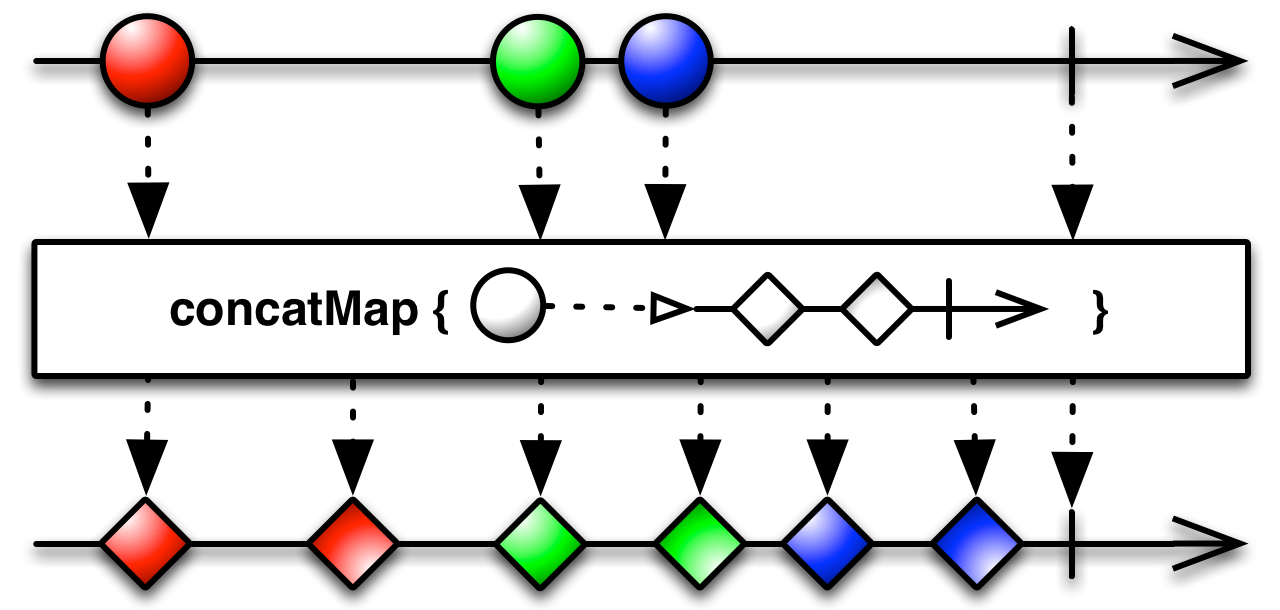### #exhaustMapSource

``exhaustMap :: forall b a. (a -> Observable b) -> Observable a -> Observable b``

It's Like concatMap (a.k.a, `>>=`) EXCEPT that it ignores every new projected ObservableImpl if the previous projected ObservableImpl has not yet completed.### #expandSource

``expand :: forall a. (a -> Observable a) -> Observable a -> Observable a``

It's similar to mergeMap, but applies the projection function to every source value as well as every output value. It's recursive.

### #groupBySource

``groupBy :: forall b a. (a -> b) -> Observable a -> Observable (Observable a)``

Groups the items emitted by an ObservableImpl (arg2) according to the value returned by the grouping function (arg1). Each group becomes its own ObservableImpl.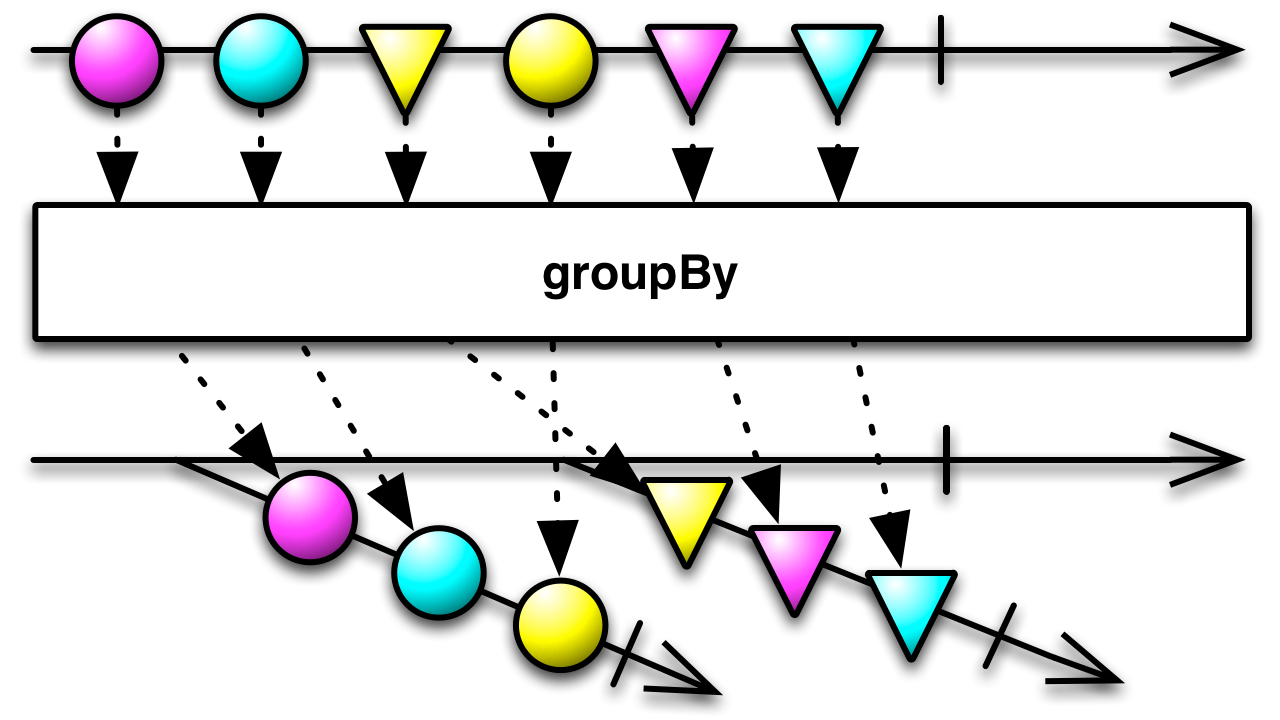### #switchMapSource

``switchMap :: forall b a. (a -> Observable b) -> Observable a -> Observable b``

Projects each source value to an ObservableImpl which is merged in the output ObservableImpl, emitting values only from the most recently projected ObservableImpl.### #delayWhenSource

``delayWhen :: forall b a. (a -> Observable b) -> Observable a -> Observable a``

Delays the emission of items from the source ObservableImpl by a given time span determined by the emissions of another ObservableImpl.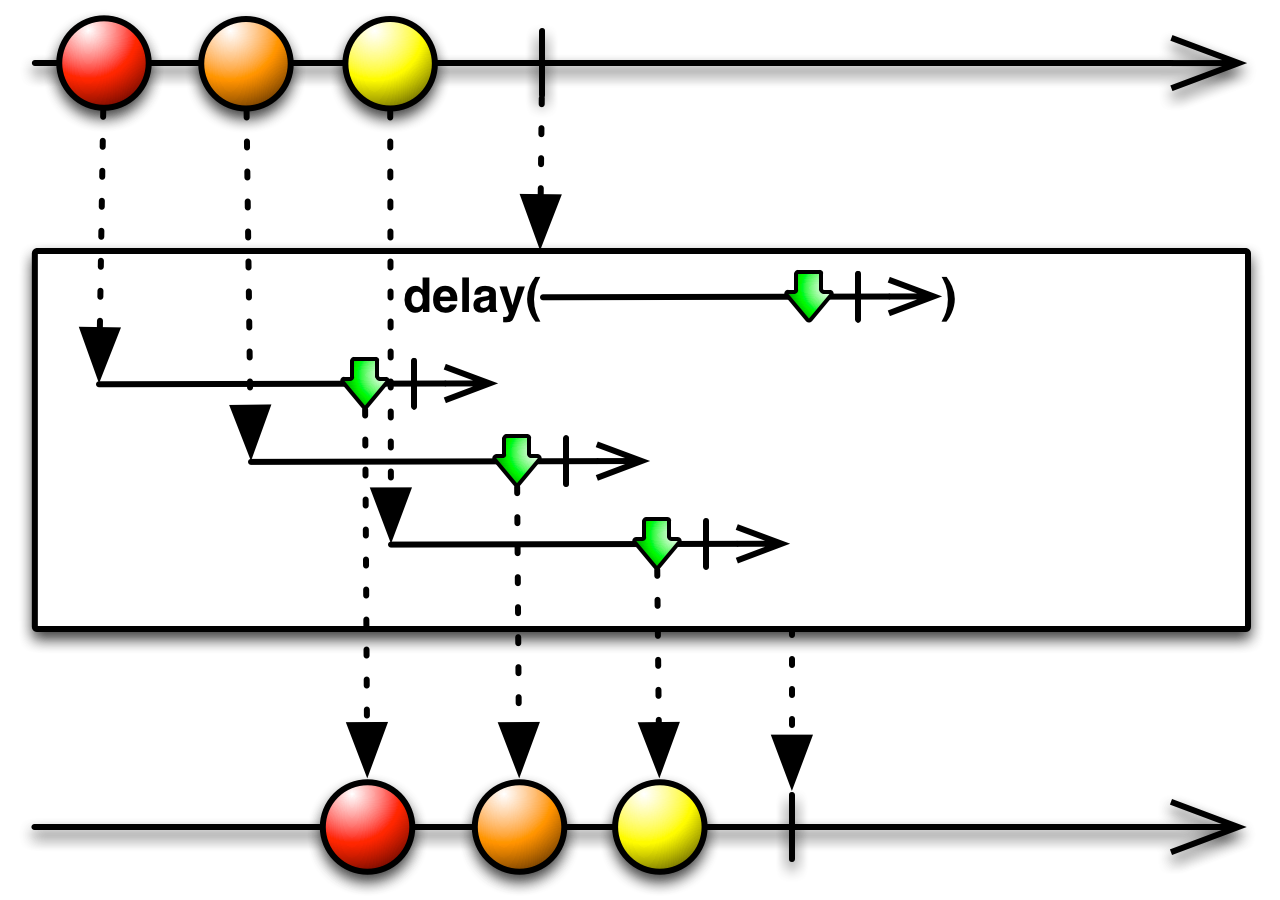### #concatAllSource

``concatAll :: forall a. Observable (Observable a) -> Observable a``

Converts a higher-order ObservableImpl into a first-order ObservableImpl by concatenating the inner ObservableImpls in order.### #mergeAllSource

``mergeAll :: forall a. Observable (Observable a) -> Observable a``

Converts a higher-order ObservableImpl into a first-order ObservableImpl which concurrently delivers all values that are emitted on the inner ObservableImpls.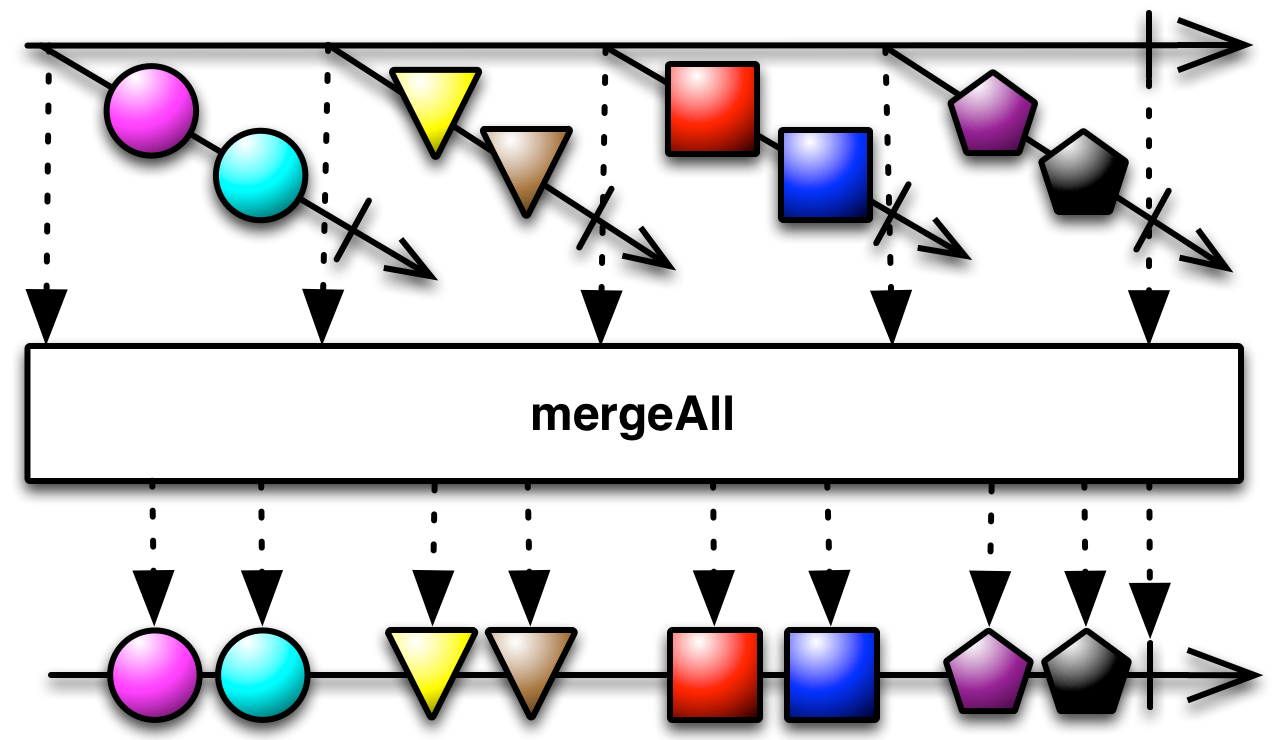### #raceSource

``race :: forall a. Array (Observable a) -> Observable a``

Returns an ObservableImpl that mirrors the first source ObservableImpl to emit an item from the array of ObservableImpls.

### #exhaustSource

``exhaust :: forall a. Observable (Observable a) -> Observable a``

Flattens an Observable-of-Observable by dropping the next inner Observables while the current inner is still executing.### #windowSource

``window :: forall b a. Observable b -> Observable a -> Observable (Observable a)``

It's like buffer, but emits a nested ObservableImpl instead of an array.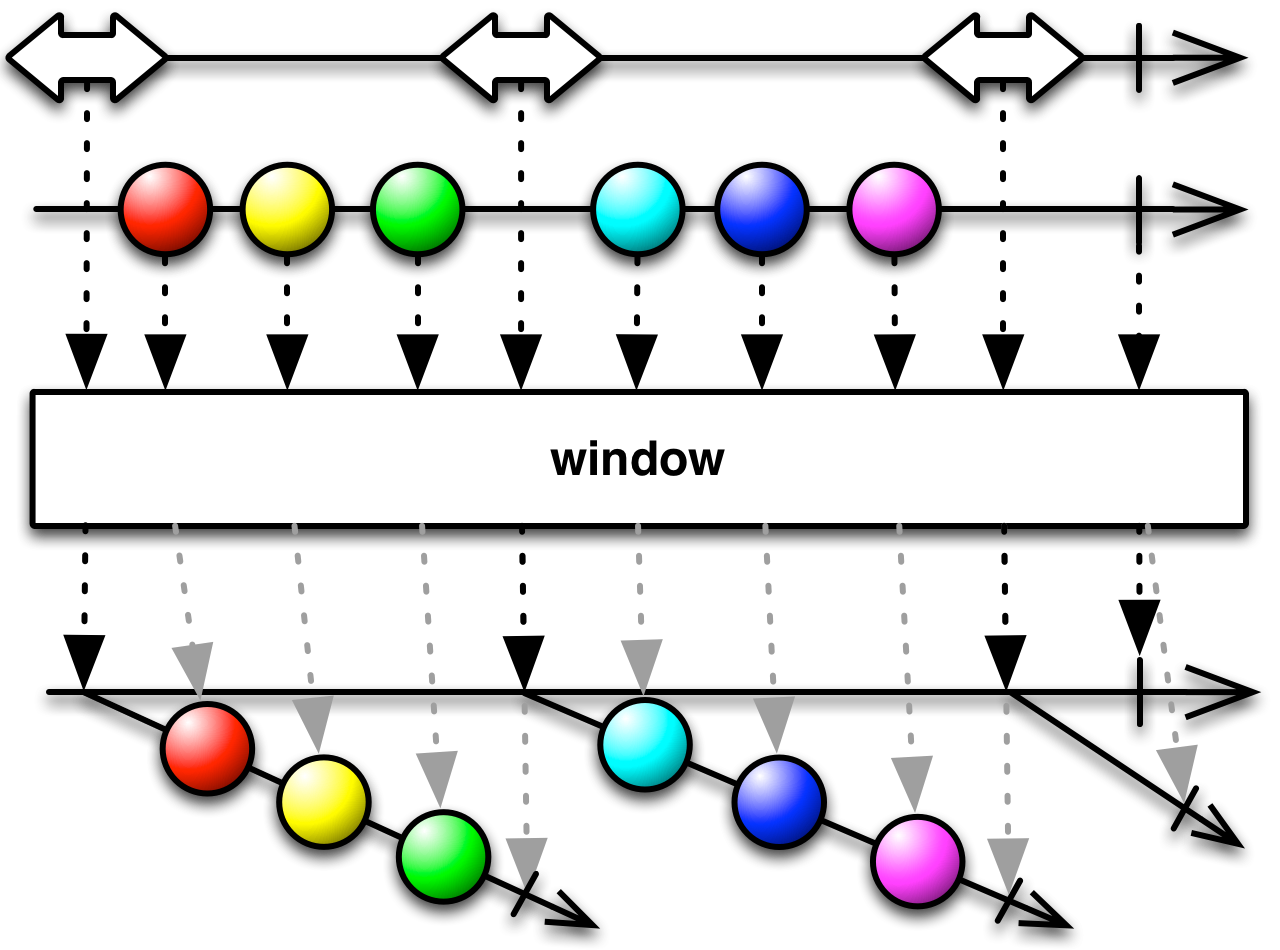### #windowCountSource

``windowCount :: forall a. Int -> Int -> Observable a -> Observable (Observable a)``

It's like bufferCount, but emits a nested ObservableImpl instead of an array.

### #windowTimeSource

``windowTime :: forall a. Int -> Int -> Observable a -> Observable (Observable a)``

It's like bufferTime, but emits a nested ObservableImpl instead of an array, and it doesn't take a maximum size parameter. arg1 is how long to buffer items into a new ObservableImpl, arg2 is the when the next buffer should begin, and arg3 is the source ObservableImpl.

### #throttleSource

``throttle :: forall b a. (a -> Observable b) -> Observable a -> Observable a``

It's like throttleTime, but the silencing duration is determined by a second ObservableImpl.### #zipSource

``zip :: forall a. Array (Observable a) -> Observable (Array a)``

Waits for each ObservableImpl to emit a value. Once this occurs, all values with the corresponding index will be emitted. This will continue until at least one inner ObservableImpl completes.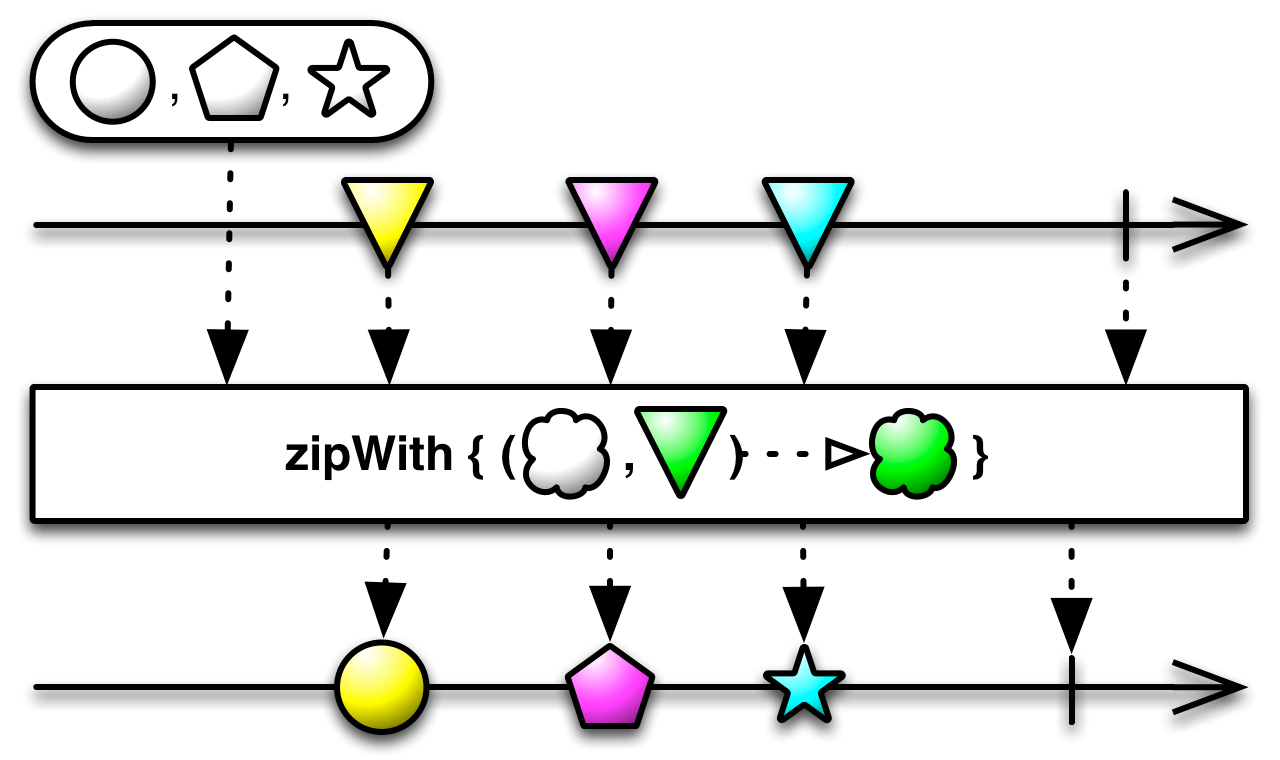### #catchSource

``catch :: forall a. Observable a -> (Error -> Observable a) -> Observable a``